In :
# Set plot options in Jupyter Lab
options(repr.plot.width = 20)
options(repr.plot.height = 6)


## Revisiting Time Series Decomposition and (S)ARMA models¶

We will revisit some of the examples for Time Series Decomposition and Seasonal ARMA model estimation

In :
?fma::airpass

 airpass {fma} R Documentation

## Monthly Airline Passenger Numbers 1949-1960

### Description

The classic Box & Jenkins airline data. Monthly totals of international airline passengers (1949–1960).

### Usage

airpass

### Format

A monthly time series, in thousands.

### Source

Makridakis, Wheelwright and Hyndman (1998) Forecasting: methods and applications, John Wiley & Sons: New York. Exercise 2.4, Chapter 3, Exercise 4.7.

### References

Box, Jenkins and Reinsell (1994) Time series analysis: forecasting and control, 3rd edition, Holden-Day: San Francisco. Series G.

### Examples

plot(airpass)
seasonplot(airpass)
tsdisplay(airpass)


[Package fma version 2.3 ]
In :
airpass <- fma::airpass


We can examine the data plot:

In :
plot.ts(airpass)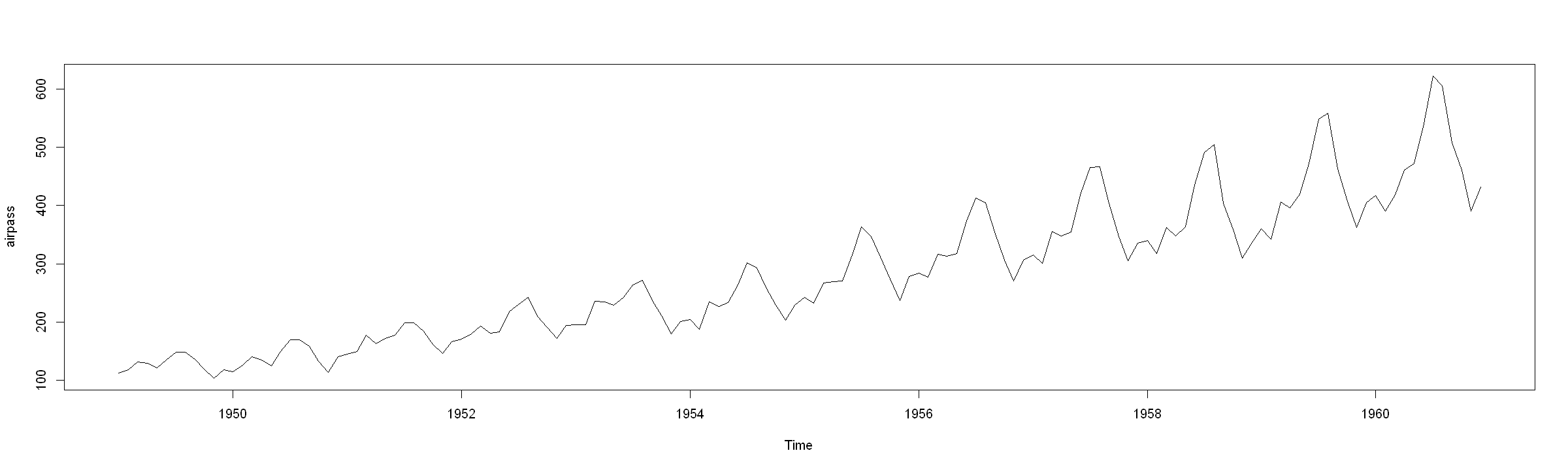Note the totak number of years in the series:

In :
total_years <- length(unique(floor(time(airpass))))
print(total_years)

 12


We can also plot the seasonal plot:

In :
forecast::seasonplot(airpass, year.labels = TRUE, year.labels.left = TRUE, col = 1:total_years)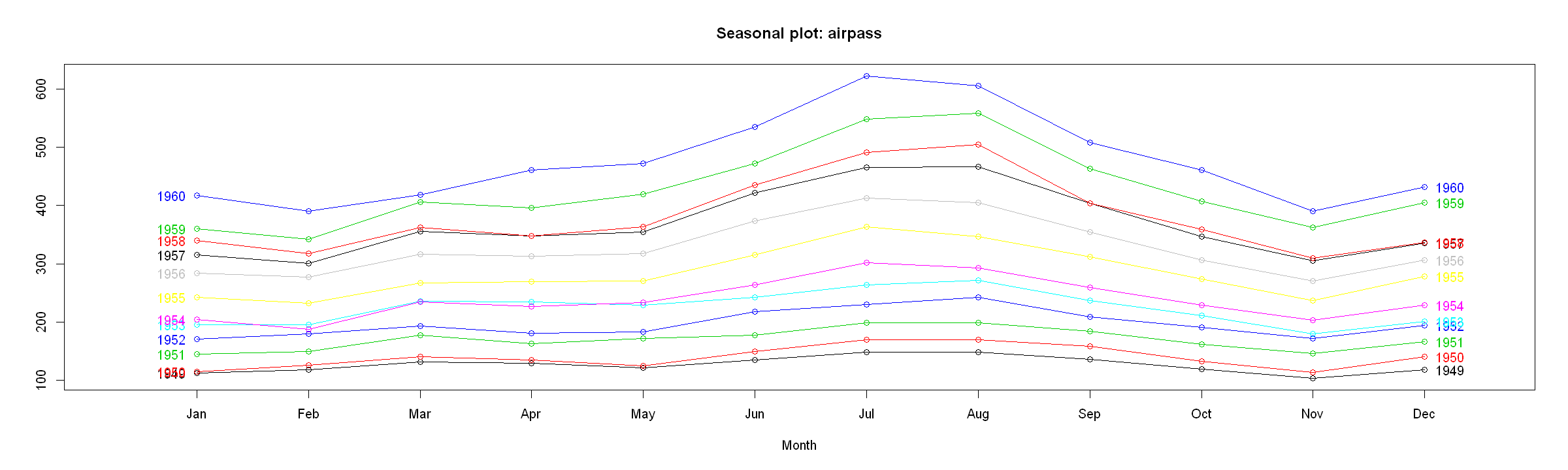Looking at the $\rm ACF$ and $\rm PACF$ plots:

In :
forecast::tsdisplay(airpass)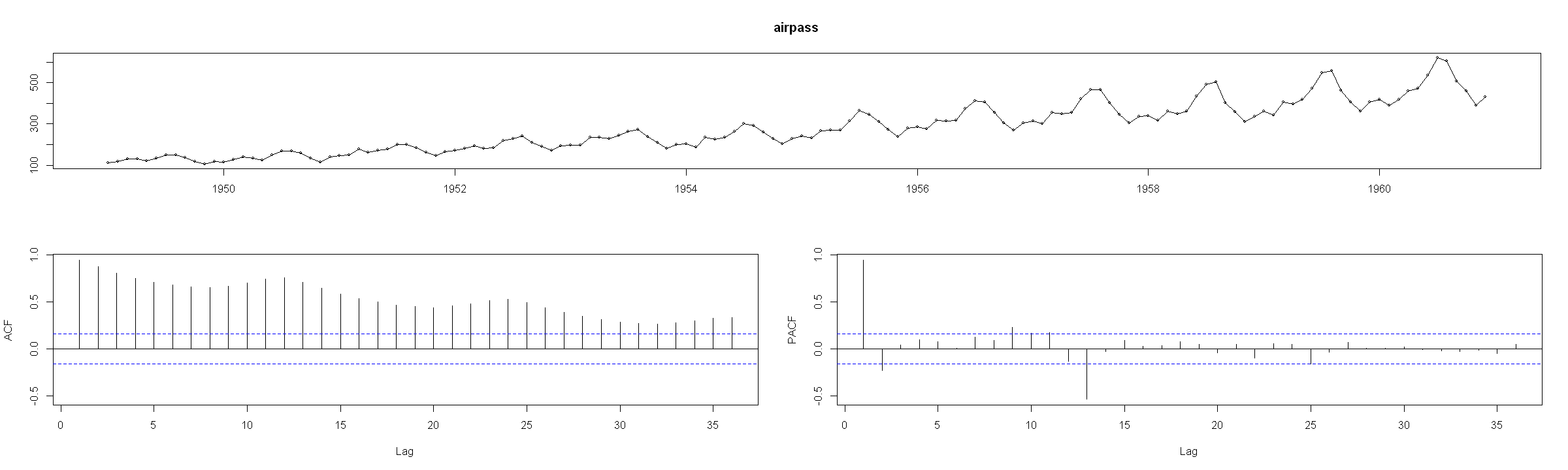and note the ACF slowly declining and the decline is in waves, which appear to repeat every 12 lags - this indicates seasonality. The time series chart indicates that there is an increasing trend.

## Time Series Transformation¶

The variation appears to be increasing, which also indicates that the series may be multiplicative: $Y_t = T_t \cdot S_t \cdot E_t$.

We want to take logarithms of the data, to get an additive form: $\log(Y_t) = \widetilde{Y}_t$ so that $\widetilde{Y}_t = \widetilde{T}_t + \widetilde{S}_t + \widetilde{E}_t$

In :
log_passengers = log(airpass)

In :
forecast::tsdisplay(log_passengers)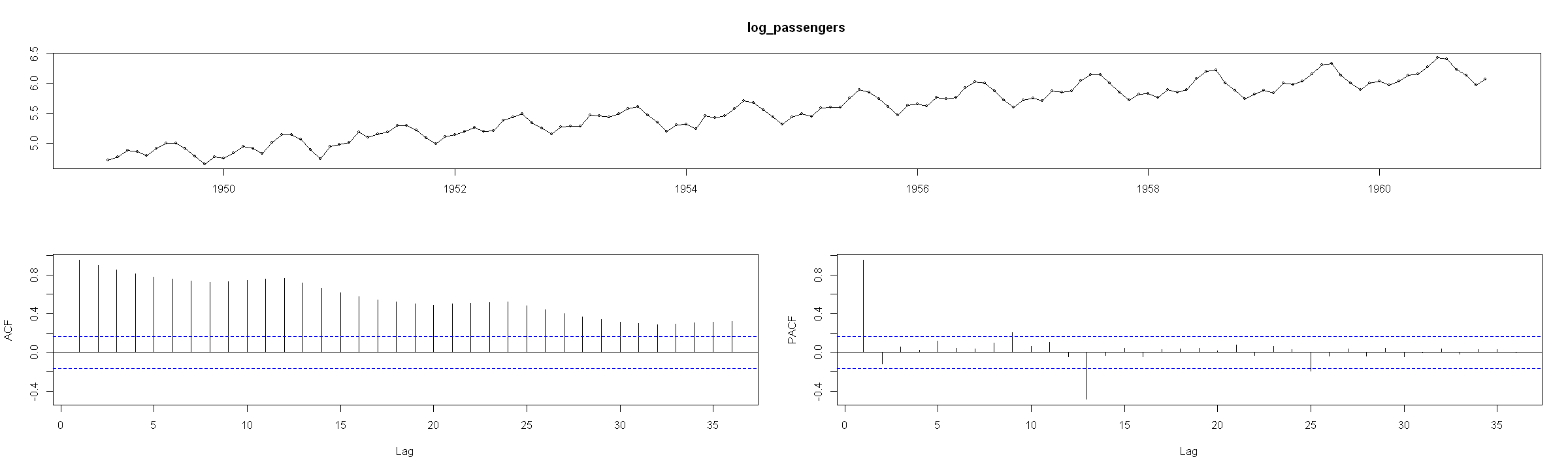Now the series appears to be additive. We can move on to decomposition of the series.

## Decomposition via Moving-average¶

### Trend Decomposition¶

We can decompose the series manually via the filter(...) function:

In :
one_sided_trd <- filter(log_passengers, filter = c(1/24, rep(1/12, total_years - 1), 1/24), sides = 1)
two_sided_trd <- filter(log_passengers, filter = c(1/24, rep(1/12, total_years - 1), 1/24), sides = 2)

In :
plot.ts(log_passengers, main = "Log of Airpassangers")
lines(one_sided_trd, col = "red")
lines(two_sided_trd, col = "blue")
legend(x = "topleft", legend = c("Actual", "One-sided MA Trend", "Two-sided MA Trend"), lty = 1, col = c("black", "red", "blue"))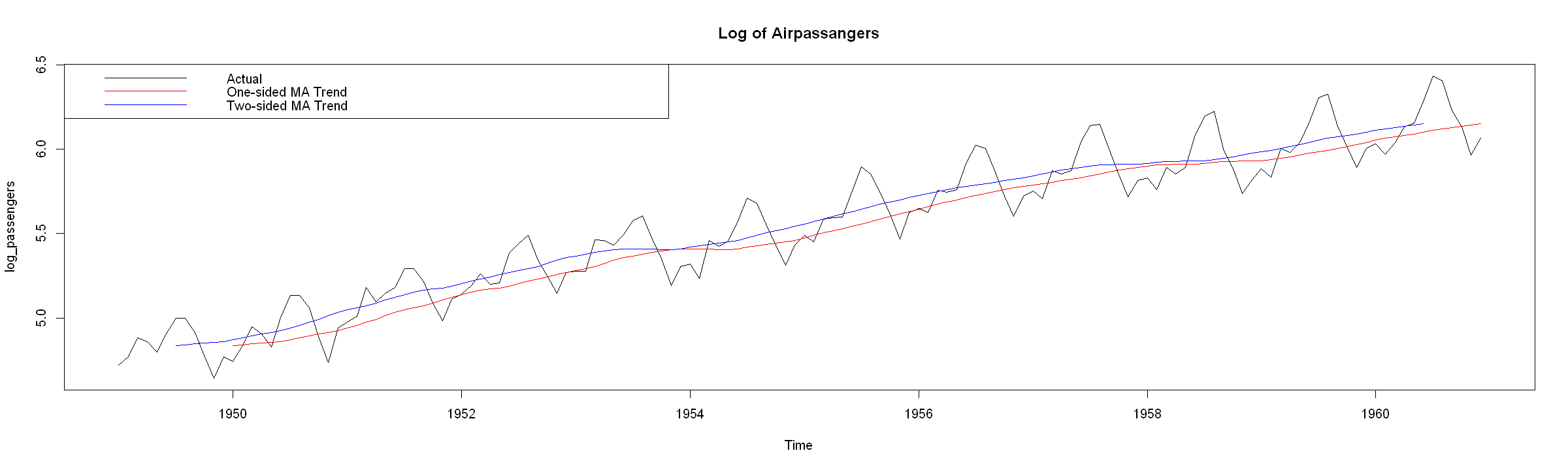### Seasonal Decomposition using the decomposed trend¶

Next, we move to decompose the seasonality

In :
#seasonal component of one-sided averaging
one_sided_season <- log_passengers - one_sided_trd
one_sided_season <- matrix(one_sided_season, ncol = 12, byrow = TRUE)
one_sided_season <- apply(one_sided_season, 2, mean, na.rm = TRUE)
#seasonal component of two-sided averaging
two_sided_season <- log_passengers - two_sided_trd
two_sided_season <- matrix(two_sided_season, ncol = 12, byrow = TRUE)
two_sided_season <- apply(two_sided_season, 2, mean, na.rm = TRUE)


We then scale the seasons to sum up to 0:

In :
one_sided_season <- one_sided_season - sum(one_sided_season) / length(one_sided_season)

In :
two_sided_season <- two_sided_season - sum(two_sided_season) / length(two_sided_season)

In :
par(mfrow = c(1, 2))
plot.ts(one_sided_season, main = "Seasonality from One-sided trend decomposition")
plot.ts(two_sided_season, main = "Seasonality from Two-sided trend decomposition")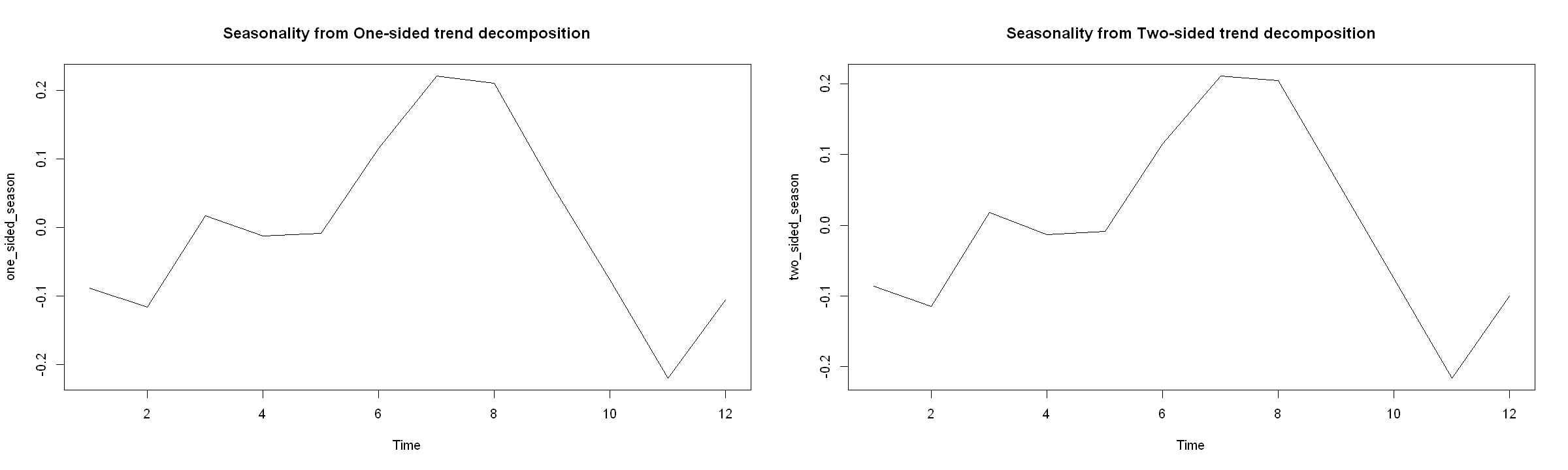Using $S_t = S_{t+d}$, create the series for the full years:

In :
one_sided_season <- rep(one_sided_season, times = total_years)
one_sided_season <- ts(one_sided_season, start = start(log_passengers), freq = frequency(log_passengers))
#
two_sided_season <- rep(two_sided_season, times = total_years)
two_sided_season <- ts(two_sided_season, start = start(log_passengers), freq = frequency(log_passengers))


Next, we can examine the decomposition visually

In :
par(mfrow = c(1, 2))
#
plot(log_passengers, lwd = 2, main = "One-sided averaging decomposition")
lines(one_sided_trd, col = "blue", lwd = 2)
lines(one_sided_trd + one_sided_season, col = "red", lwd = 2)
#
plot(log_passengers, lwd = 2, main = "Two-sided averaging decomposition")
lines(two_sided_trd, col = "blue", lwd = 2)
lines(two_sided_trd + two_sided_season, col = "red", lwd = 2)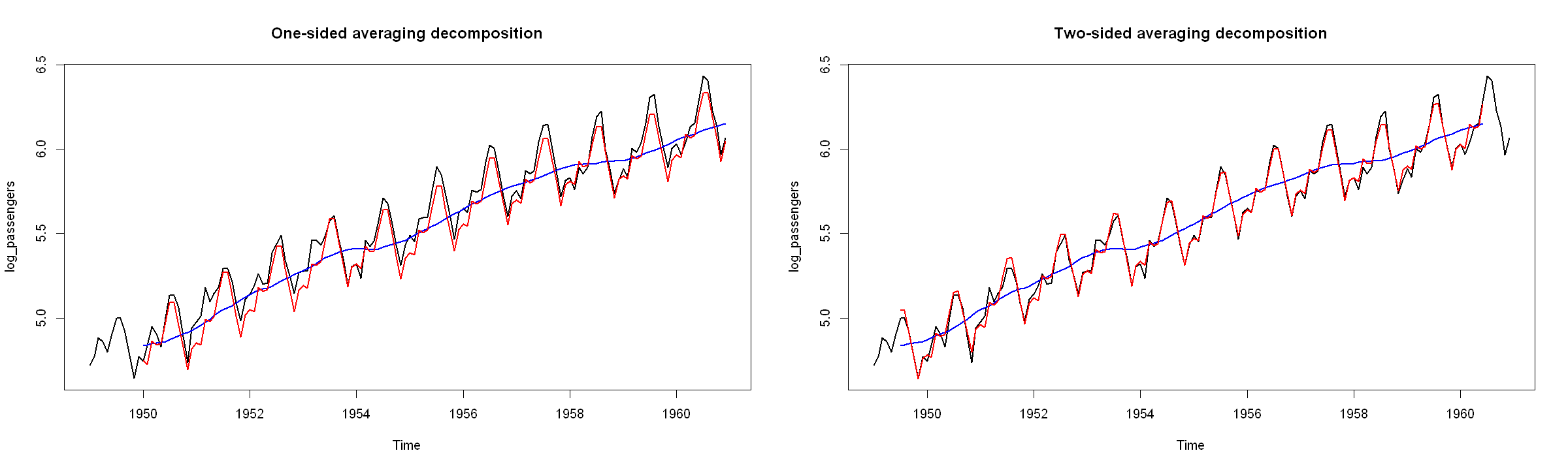We can also use the decompose(...) function to do all of this:

In :
two_sided_decomp <- decompose(log_passengers, type = "additive")


Compared with the filter() function:

In :
two_sided_trd - two_sided_decomp$trend   Jan Feb Mar Apr May Jun Jul Aug Sep Oct Nov Dec 1949 NA NA NA NA NA NA 0 0 0 0 0 0 1950 0 0 0 0 0 0 0 0 0 0 0 0 1951 0 0 0 0 0 0 0 0 0 0 0 0 1952 0 0 0 0 0 0 0 0 0 0 0 0 1953 0 0 0 0 0 0 0 0 0 0 0 0 1954 0 0 0 0 0 0 0 0 0 0 0 0 1955 0 0 0 0 0 0 0 0 0 0 0 0 1956 0 0 0 0 0 0 0 0 0 0 0 0 1957 0 0 0 0 0 0 0 0 0 0 0 0 1958 0 0 0 0 0 0 0 0 0 0 0 0 1959 0 0 0 0 0 0 0 0 0 0 0 0 1960 0 0 0 0 0 0 NA NA NA NA NA NA The results are the same. The upside is we automatically get the seasonal decomposition as well, which are the same as our manually calculated ones: In : two_sided_season - two_sided_decomp$seasonal

     Jan Feb Mar Apr May Jun Jul Aug Sep Oct Nov Dec
1949   0   0   0   0   0   0   0   0   0   0   0   0
1950   0   0   0   0   0   0   0   0   0   0   0   0
1951   0   0   0   0   0   0   0   0   0   0   0   0
1952   0   0   0   0   0   0   0   0   0   0   0   0
1953   0   0   0   0   0   0   0   0   0   0   0   0
1954   0   0   0   0   0   0   0   0   0   0   0   0
1955   0   0   0   0   0   0   0   0   0   0   0   0
1956   0   0   0   0   0   0   0   0   0   0   0   0
1957   0   0   0   0   0   0   0   0   0   0   0   0
1958   0   0   0   0   0   0   0   0   0   0   0   0
1959   0   0   0   0   0   0   0   0   0   0   0   0
1960   0   0   0   0   0   0   0   0   0   0   0   0

We can also plot the series:

In :
plot.ts(log_passengers, main = "Log of Airpassangers")
lines(two_sided_decomp$trend, col = "blue", lwd = 2) lines(two_sided_decomp$trend + two_sided_decomp$seasonal, col = "red", lwd = 2)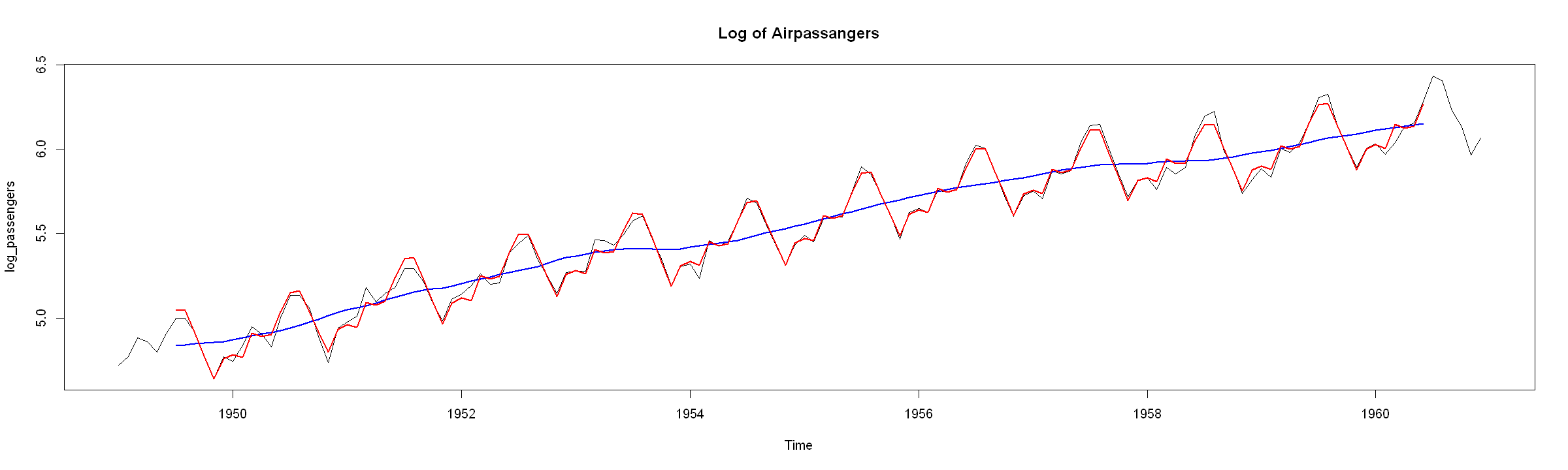and examine the residuals: In : forecast::tsdisplay(two_sided_decomp$random)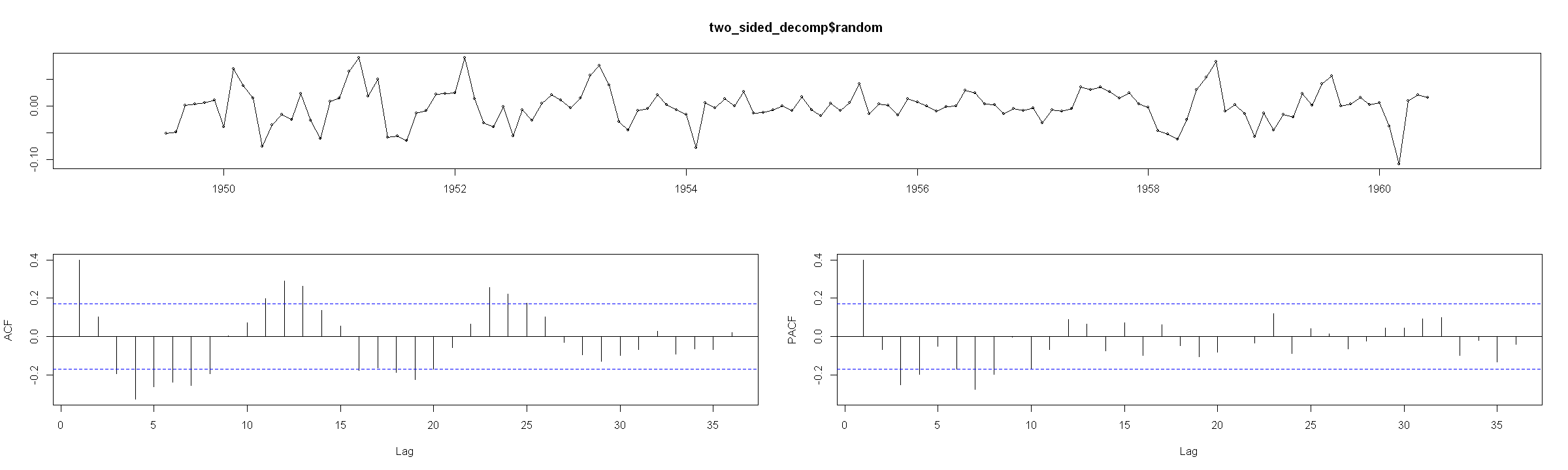Looking at the $\rm ACF$, the residuals still appear to have some searonality.

## Exponential Smoothing examples¶

### Simple exponential smoothing:¶

In :
s_es <- forecast::ses(log_passengers)

In :
par(mfrow = c(1, 2))
#
plot.ts(log_passengers)#, main = "Simple Exponential smoothing")
lines(fitted.values(s_es), col = "blue", lty = 2, lwd = 2)
lines(fitted.values(forecast::ses(log_passengers, alpha = 0.1)), col = "darkorange", lty = 2, lwd = 2)
#
plot(forecast::forecast(s_es, h = 10))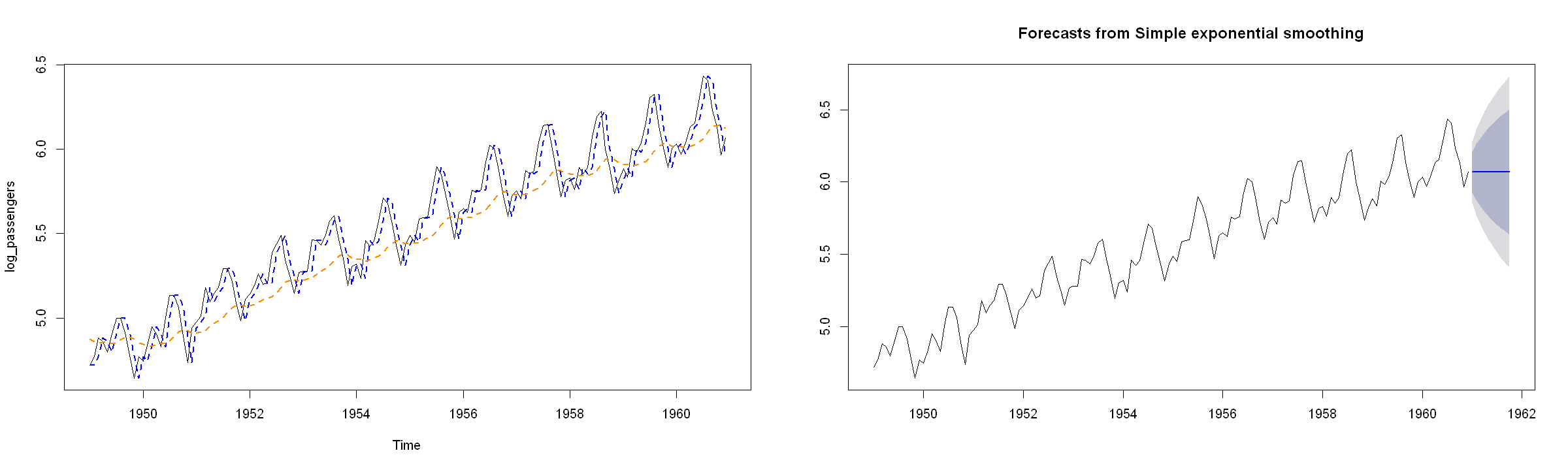### Double Exponential Smoothing (Holt's Linear Method)¶

In :
d_es <- forecast::holt(log_passengers)

In :
par(mfrow = c(1, 2))
#
plot.ts(log_passengers)#, main = "Double Exponential smoothing")
lines(fitted.values(d_es), col = "blue", lty = 2, lwd = 2)
lines(fitted.values(forecast::holt(log_passengers, alpha = 0.1, beta = 0.09)), col = "darkorange", lty = 2, lwd = 2)
#
plot(forecast::forecast(d_es, h = 10))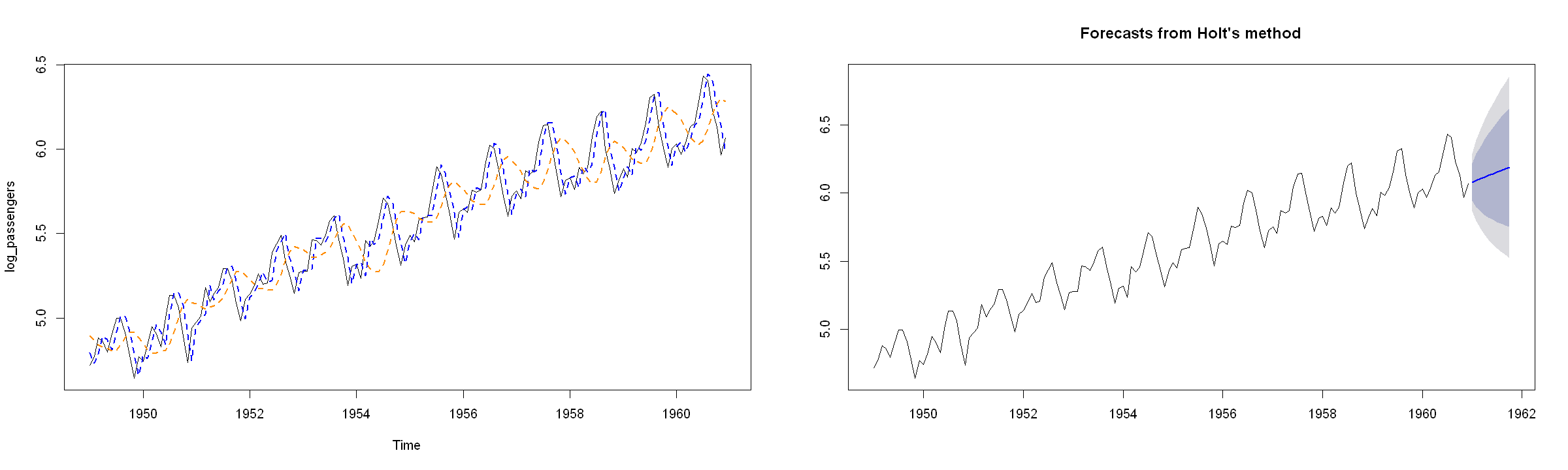### Triple Exponential Smoothing (Holt-Winter's Method)¶

In :
t_es <- forecast::hw(log_passengers)

In :
par(mfrow = c(1, 2))
#
plot.ts(log_passengers)#, main = "Triple Exponential smoothing")
lines(fitted.values(t_es), col = "blue", lty = 2, lwd = 2)
#
plot(forecast::forecast(t_es, h = 10))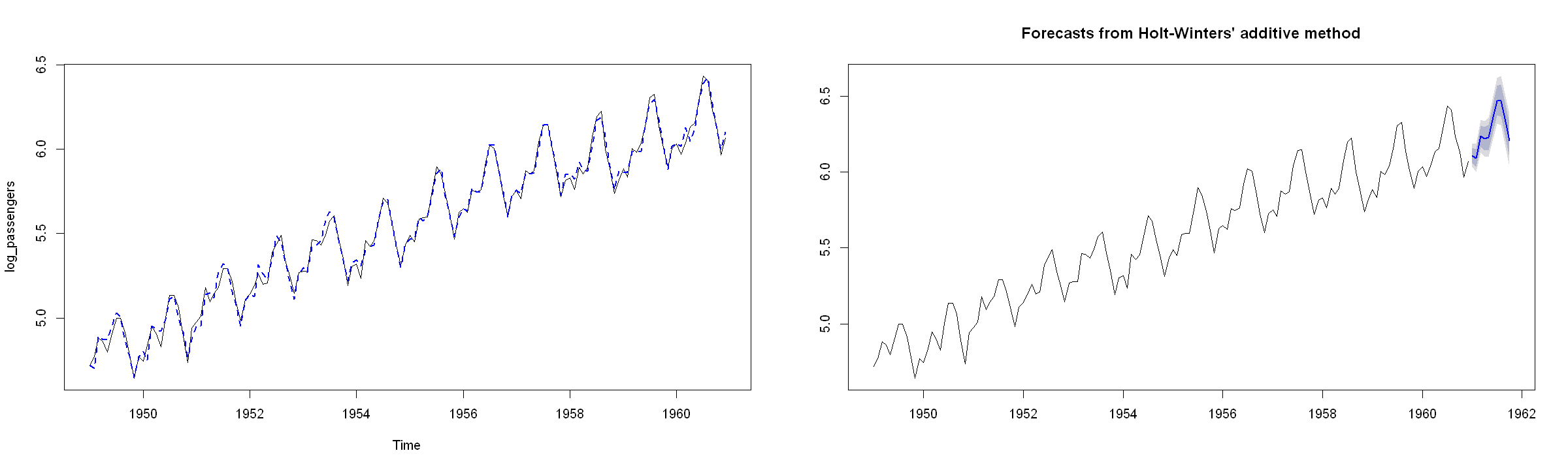R allows to use an automated procedure to choose the smoothing parameters - the function ets selects the best variant automatically (by the minimum of AIC) and estimates its parameters:

In :
ets_es <- forecast::ets(log_passengers)

In :
par(mfrow = c(1, 2))
#
plot.ts(log_passengers)
lines(fitted.values(ets_es), col = "blue", lty = 2, lwd = 2)
#
plot(forecast::forecast(ets_es, h = 10))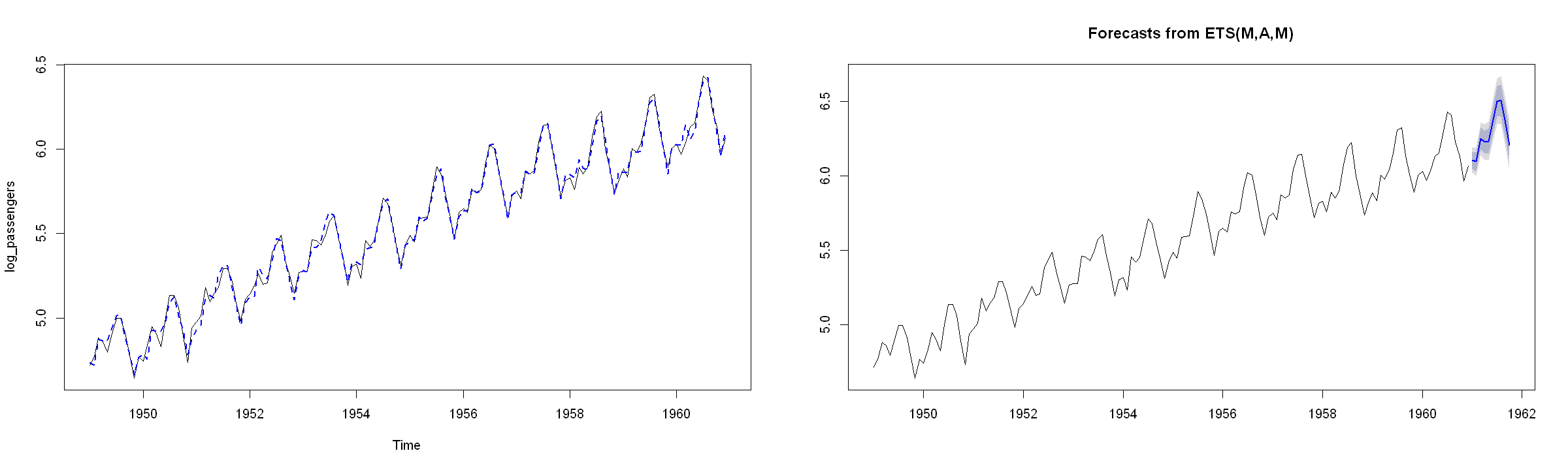Note the help file:

• The first letter denotes the error type ("A", "M" or "Z");
• The second letter denotes the trend type ("N","A","M" or "Z");
• The third letter denotes the season type ("N","A","M" or "Z").

In all cases, "N"=none, "A"=additive, "M"=multiplicative and "Z"=automatically selected. So, for example, "ANN" is simple exponential smoothing with additive errors, "MAM" is multiplicative Holt-Winters' method with multiplicative errors, and so on.

In :
forecast::tsdisplay(ets_es$residuals)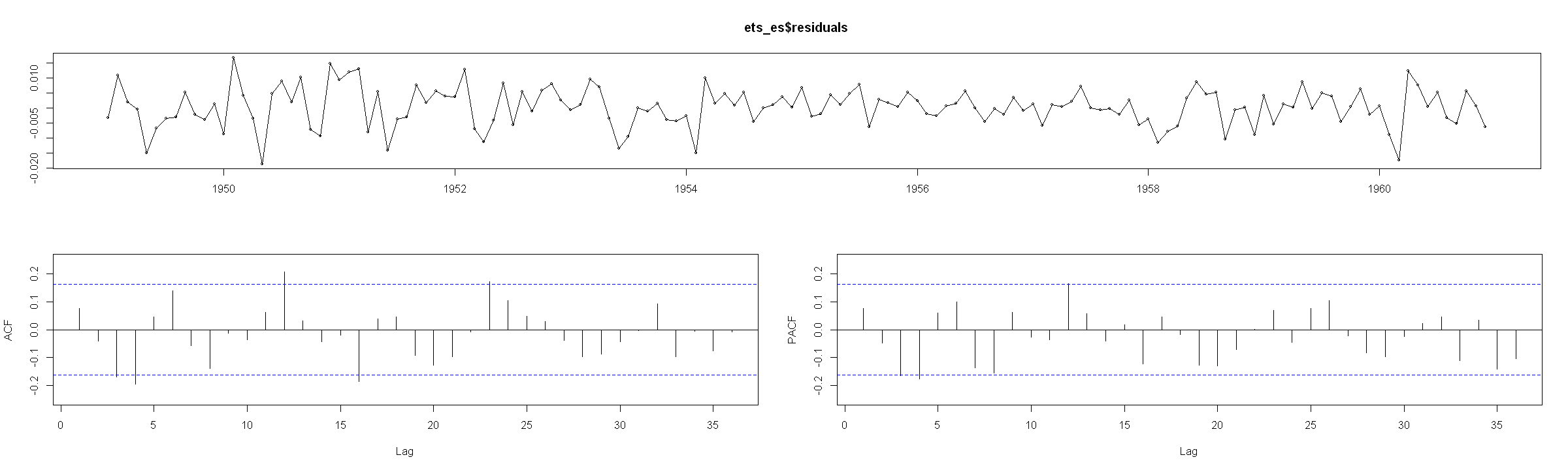The residuals ppear to have a slight autocorrelation ## SARMA models with trend¶ In : tt_dt <- data.frame(tt = 1:length(log_passengers)) tt_dt$tt_sq <- tt_dt$tt^2 # mdl_auto = forecast::auto.arima(airpass, max.d = 0, max.D = 0, xreg = tt_dt)  In : mdl_auto  Series: airpass Regression with ARIMA(0,0,3)(0,0,1) errors Coefficients: ma1 ma2 ma3 sma1 intercept tt tt_sq 0.9875 0.7195 0.3621 0.7592 117.1154 1.5895 0.0068 s.e. 0.0822 0.1077 0.0914 0.0668 20.7197 0.6575 0.0044 sigma^2 estimated as 359.3: log likelihood=-630.18 AIC=1276.35 AICc=1277.42 BIC=1300.11 In : plot(airpass, main = "SARMA with trend") lines(mdl_auto$fitted, col = "red")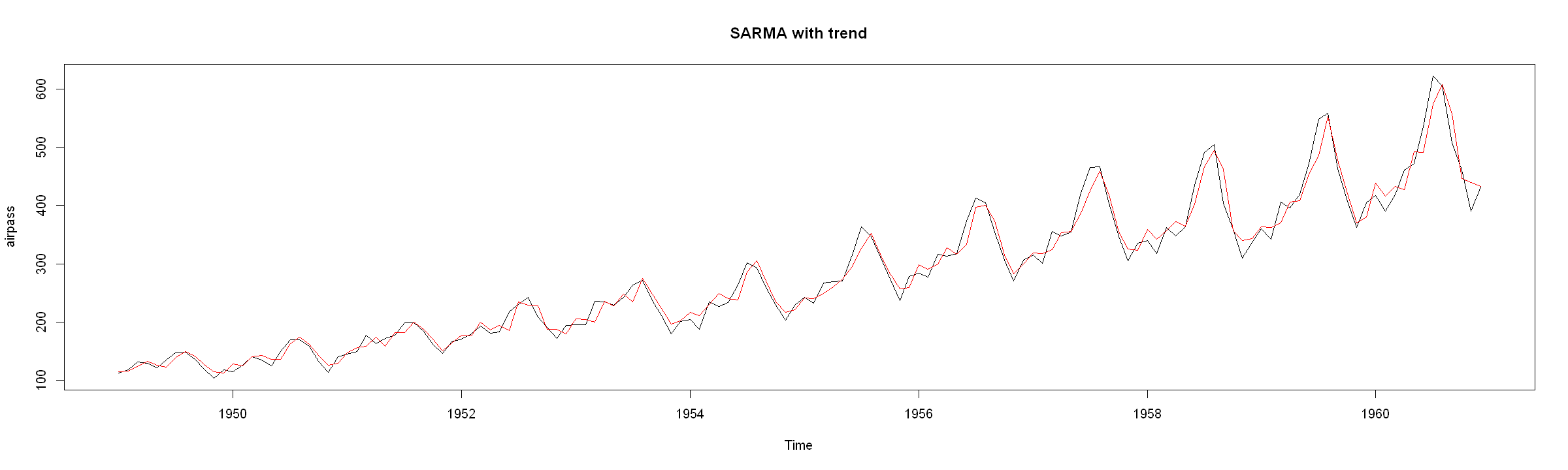We can examine the residuals

In :
forecast::tsdisplay(mdl_auto$residuals)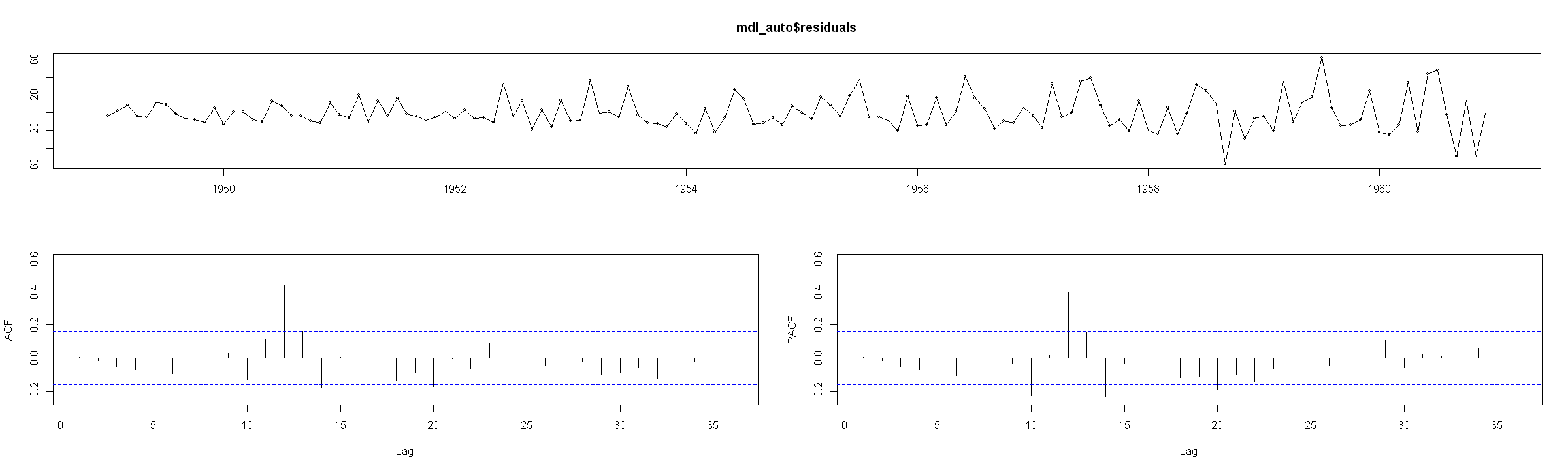Note that there appears to be a large autocorrelation for every 12-th lag. So the restriction of no differencing causes auto.arima to fail to find a suitible model. For somparison, if we were to specify a$SARIMA(1, 0, 1)\times(1, 0, 1)_{12}$. If we use the default specification, we may get a warning that the series is not stationary: In : tryCatch({ forecast::Arima(airpass, order = c(1, 0, 1), seasonal = c(1, 0, 1), xreg = tt_dt) }, warning = function(w) { # warning-handler-code }, error = function(e) { print(e) }, finally = { # cleanup-code })  <simpleError in stats::arima(x = x, order = order, seasonal = seasonal, xreg = xreg, include.mean = include.mean, method = method, ...): non-stationary seasonal AR part from CSS>  If we are sure that the process is stationary, we can specify a different parameter estimation method: In : mdl_manual = forecast::Arima(airpass, order = c(1, 0, 1), seasonal = c(1, 0, 1), xreg = tt_dt, method = "ML")  In : mdl_manual  Series: airpass Regression with ARIMA(1,0,1)(1,0,1) errors Coefficients: ar1 ma1 sar1 sma1 intercept tt tt_sq 0.7540 -0.1003 0.9630 -0.1336 119.0055 1.4857 0.0077 s.e. 0.0724 0.1045 0.0161 0.0925 38.2025 0.5299 0.0033 sigma^2 estimated as 128: log likelihood=-564.67 AIC=1145.35 AICc=1146.41 BIC=1169.11 In : plot(airpass, main = "SARMA with trend") lines(mdl_manual$fitted, col = "red")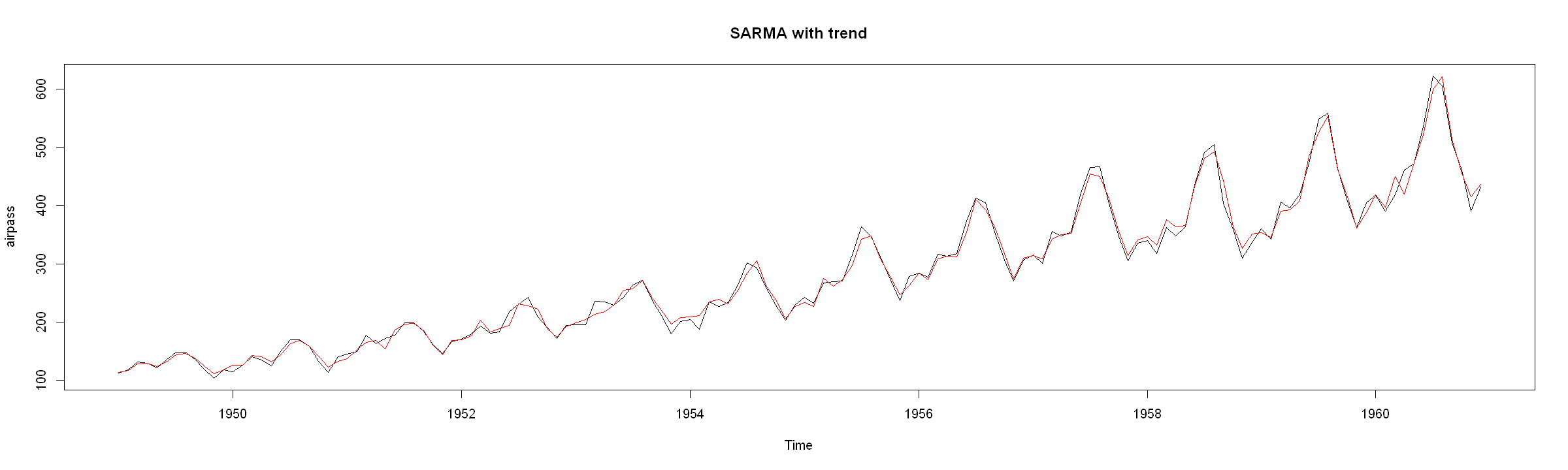In :
forecast::tsdisplay(mdl_manual$residuals)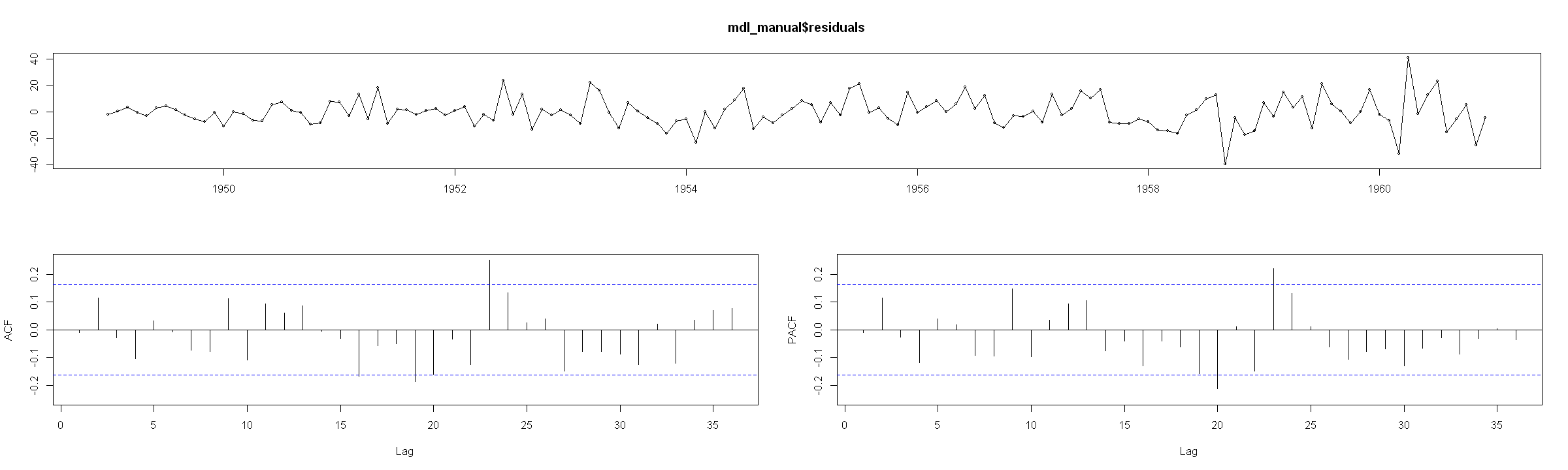The residuals look much better compared to the auto.arima results with restriction that the data is not to be differenced. Finally, we can forecast the process. we do need to have the soem future values of the trend. Thankfully, we have opted to use the standard indexing of$t = 1,2,...$for the trend. In : tt_forc <- data.frame(tt = tail(tt_dt$tt, 1) + 1:20)
tt_forc$tt_sq <- tt_forc$tt^2

In :
plot(forecast::forecast(mdl_manual, xreg = tt_forc))
lines(mdl_manual$fitted, col = "red")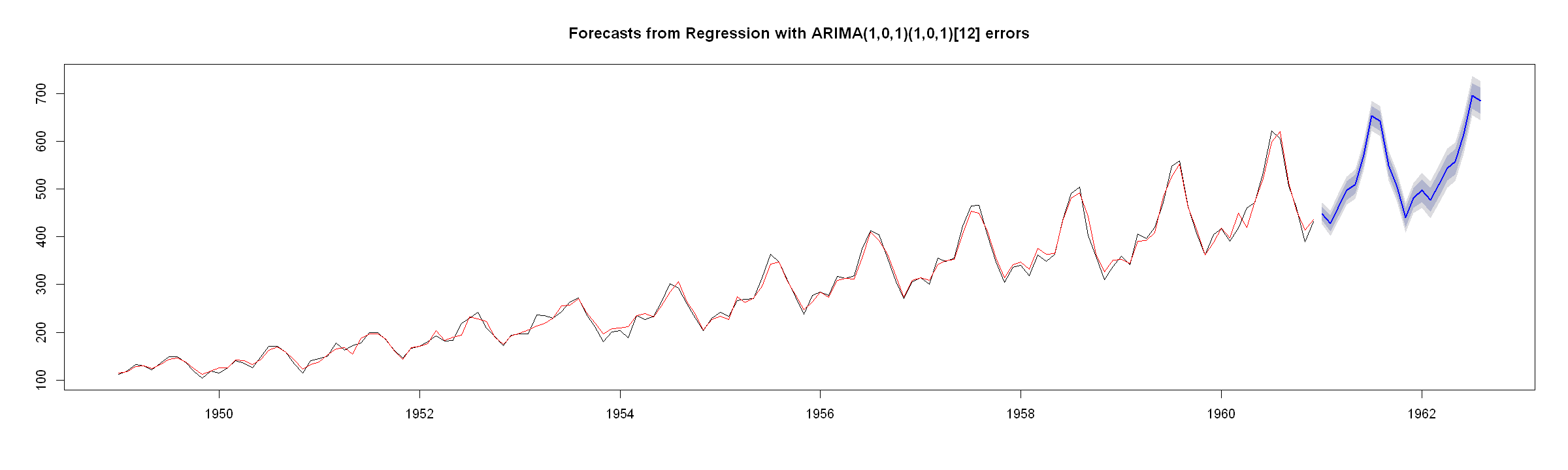# Time series with unit root¶ In : # Set plot options in Jupyter Lab options(repr.plot.width = 15) options(repr.plot.height = 4)  # (1) Series plot and transformation¶ In : suppressPackageStartupMessages({library(forecast)})  In : nyse <- read.table(url("http://uosis.mif.vu.lt/~rlapinskas/(data%20R&GRETL/nyse.txt"), header = TRUE) nyse <- ts(nyse, start = 1952, frequency = 12)  In : head(nyse, 36)   Jan Feb Mar Apr May Jun Jul 1952 100.00000 101.55801 98.35478 102.49584 97.45797 99.92923 103.53153 1953 107.99719 107.70146 106.74088 104.92693 101.94905 101.89004 99.83214 1954 102.88020 108.08621 109.38209 113.14183 117.91136 120.87454 121.79292 Aug Sep Oct Nov Dec 1952 104.61471 103.15134 100.79363 100.10055 105.16128 1953 102.22141 97.10542 96.98586 101.49628 103.35702 1954 127.93562 124.25727 132.08256 129.77409 141.50459 In : par(mfrow = c(1, 3)) plot(nyse, main = bquote(Y[t])) plot(log(nyse), main = bquote(log(Y[t]))) plot(diff(log(nyse)), main = bquote(Delta~log(Y[t])))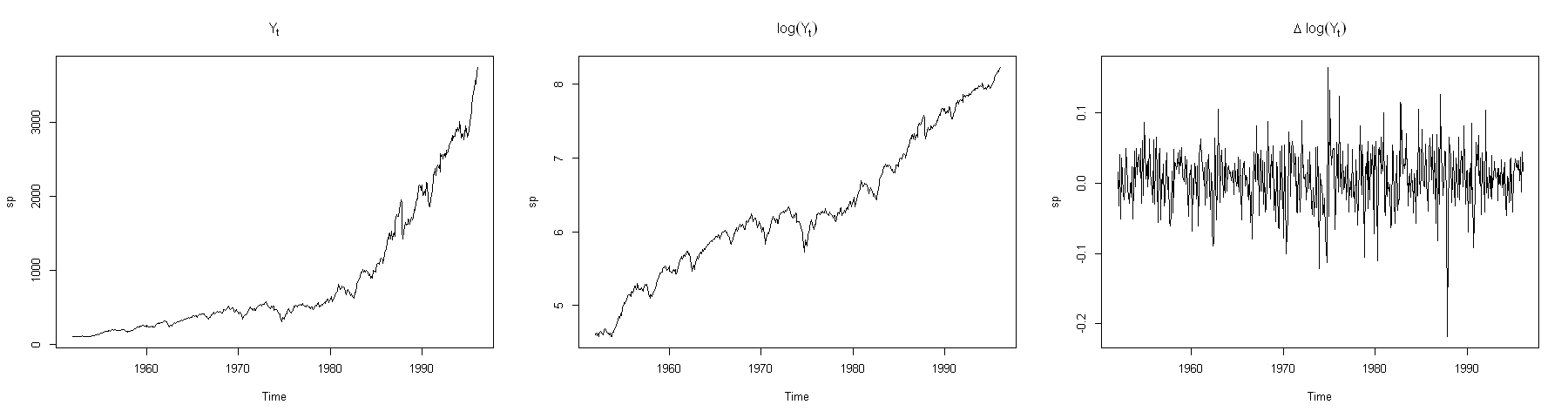Note that the growth appears to be exponential - it is more convenient to take logarithms to linearize the series. In : lnyse <- log(nyse)  # (2)$\rm ACF$and$\rm PACF$plots¶ In : par(mfrow = c(1, 2)) forecast::Acf(lnyse, ) forecast::Pacf(lnyse)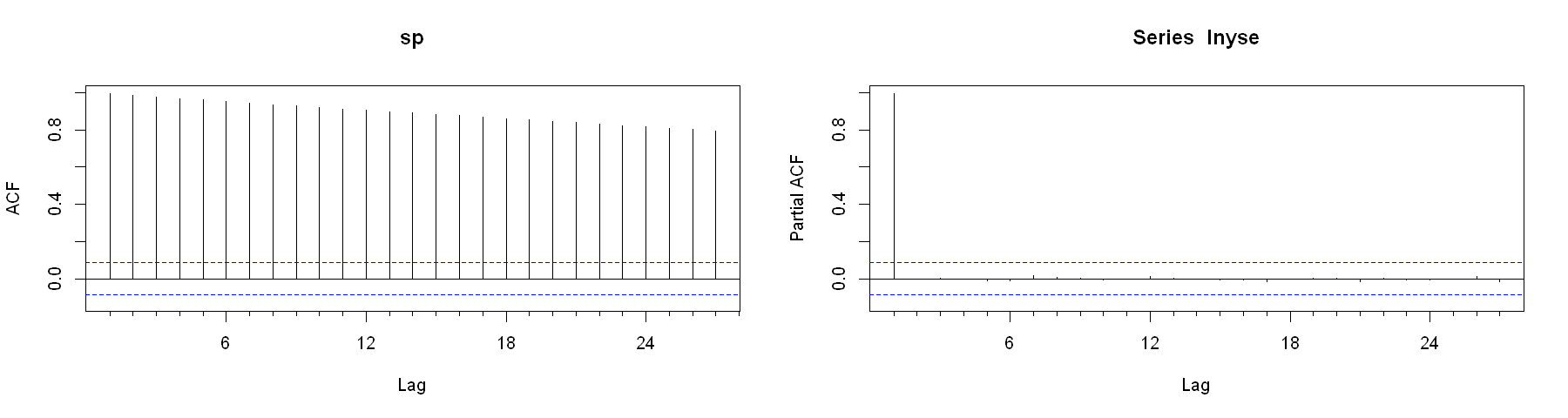Slow ACF decay - indication of non-stationarity, or a trend, or a nonstationarity and/or drift and/or trend. We need to test whether the data is TS or DS. To do this - we need to carry out unit root testing. We can always load the relevant libraries to avoid the use of :: notation. # (3) Unit root testing¶ In : library(forecast) library(dynlm) library(urca)  Loading required package: zoo Attaching package: 'zoo' The following objects are masked from 'package:base': as.Date, as.Date.numeric  ## (3.1) Manual Unit root testing¶ Unit root tests consist of: • Selecting the lag order of the autoregressive model$\Delta Y_t = \alpha + \delta t + \rho Y_{t-1} + \sum_{j = 1}^p \gamma_j \Delta Y_{t-1} + \epsilon_t$• Determine whether the trend coefficient,$\widehat{\delta}$, is significant; • Dependin on the final model, asses whether$\widehat{\rho}$is significant. ### Lag Order selection¶ We will examine three cases: • Starting with$p_\max = 4$; • Starting with$p_\max = 5$; • Starting with$p_\max = \biggr\lfloor 12 \cdot \left( \dfrac{T}{100} \right)^{1/4} \biggr\rfloor$; #### Starting with$p_\max = 4$¶ In : mod.1 <- dynlm(d(lnyse) ~ L(lnyse) + L(d(lnyse), 1:4) + time(lnyse)) summary(mod.1)  Time series regression with "ts" data: Start = 1952(6), End = 1995(13) Call: dynlm(formula = d(lnyse) ~ L(lnyse) + L(d(lnyse), 1:4) + time(lnyse)) Residuals: Min 1Q Median 3Q Max -0.220902 -0.024758 0.001637 0.026627 0.152618 Coefficients: Estimate Std. Error t value Pr(>|t|) (Intercept) -2.3687707 1.1485800 -2.062 0.0397 * L(lnyse) -0.0172567 0.0083590 -2.064 0.0395 * L(d(lnyse), 1:4)1 0.0544182 0.0439376 1.239 0.2161 L(d(lnyse), 1:4)2 -0.0282788 0.0439795 -0.643 0.5205 L(d(lnyse), 1:4)3 0.0150921 0.0439341 0.344 0.7313 L(d(lnyse), 1:4)4 0.0364522 0.0439285 0.830 0.4070 time(lnyse) 0.0012584 0.0006077 2.071 0.0389 * --- Signif. codes: 0 '***' 0.001 '**' 0.01 '*' 0.05 '.' 0.1 ' ' 1 Residual standard error: 0.04061 on 517 degrees of freedom Multiple R-squared: 0.01244, Adjusted R-squared: 0.0009837 F-statistic: 1.086 on 6 and 517 DF, p-value: 0.3696  The last lag coefficient, L(d(lnyse), 1:4)4 is insignificant since its$p-value = 0.4070 > 0.05. We will reduce the maximum lag and continue the procedure.

In :
mod.1 <- dynlm(d(lnyse) ~ L(lnyse) + L(d(lnyse), 1:3) + time(lnyse))
print(round(coef(summary(mod.1)), 4))

                  Estimate Std. Error t value Pr(>|t|)
(Intercept)        -2.2563     1.1441 -1.9721   0.0491
L(lnyse)           -0.0162     0.0083 -1.9420   0.0527
L(d(lnyse), 1:3)1   0.0514     0.0439  1.1689   0.2430
L(d(lnyse), 1:3)2  -0.0274     0.0439 -0.6227   0.5338
L(d(lnyse), 1:3)3   0.0151     0.0439  0.3438   0.7311
time(lnyse)         0.0012     0.0006  1.9789   0.0484


p-value of L(d(lnyse), 1:3)3 is > 0.05.

In :
mod.1 <- dynlm(d(lnyse) ~ L(lnyse) + L(d(lnyse), 1:2) + time(lnyse))
print(round(coef(summary(mod.1)), 4))

                  Estimate Std. Error t value Pr(>|t|)
(Intercept)        -2.2314     1.1372 -1.9621   0.0503
L(lnyse)           -0.0161     0.0083 -1.9483   0.0519
L(d(lnyse), 1:2)1   0.0493     0.0438  1.1244   0.2614
L(d(lnyse), 1:2)2  -0.0263     0.0439 -0.6004   0.5485
time(lnyse)         0.0012     0.0006  1.9698   0.0494


p-value of L(d(lnyse), 1:2)2 is > 0.05.

In :
mod.1 <- dynlm(d(lnyse) ~ L(lnyse) + L(d(lnyse), 1) + time(lnyse))
print(round(coef(summary(mod.1)), 4))

               Estimate Std. Error t value Pr(>|t|)
(Intercept)     -2.2880     1.1308 -2.0233   0.0435
L(lnyse)        -0.0164     0.0082 -1.9956   0.0465
L(d(lnyse), 1)   0.0480     0.0438  1.0956   0.2737
time(lnyse)      0.0012     0.0006  2.0302   0.0428


p-value of L(d(lnyse), 1) is > 0.05.

In :
mod.1 <- dynlm(d(lnyse) ~ L(lnyse) + time(lnyse))
print(round(coef(summary(mod.1)), 4))

            Estimate Std. Error t value Pr(>|t|)
(Intercept)  -2.1695     1.1246 -1.9292   0.0542
L(lnyse)     -0.0155     0.0082 -1.8995   0.0580
time(lnyse)   0.0012     0.0006  1.9362   0.0534


The final model contains no lags of $\Delta Y_{t-j}$ - so there is no autoregressive coefficient for the difference model.

#### Starting with $p_\max = 5$¶

We will repeat the procedure as before.

In :
mod.2 <- dynlm(d(lnyse) ~ L(lnyse) + L(d(lnyse), 1:5) + time(lnyse))
print(round(coef(summary(mod.2)), 4))

                  Estimate Std. Error t value Pr(>|t|)
(Intercept)        -2.6325     1.1505 -2.2882   0.0225
L(lnyse)           -0.0192     0.0084 -2.2920   0.0223
L(d(lnyse), 1:5)1   0.0540     0.0439  1.2319   0.2185
L(d(lnyse), 1:5)2  -0.0286     0.0439 -0.6523   0.5145
L(d(lnyse), 1:5)3   0.0208     0.0439  0.4738   0.6359
L(d(lnyse), 1:5)4   0.0329     0.0438  0.7516   0.4527
L(d(lnyse), 1:5)5   0.1030     0.0438  2.3508   0.0191
time(lnyse)         0.0014     0.0006  2.2962   0.0221


L(d(lnyse), 1:5)5 has p-value < 0.05, so the final lag order is 5.

#### Starting with $p_\max = \biggr\lfloor 12 \cdot \left( \dfrac{T}{100} \right)^{1/4} \biggr\rfloor$¶

The maximum lag via the rule-of-thumb is:

In :
floor(12 * (length(lnyse) / 100)^(1/4))

18
In :
mod.3 <- dynlm(d(lnyse) ~ L(lnyse) + L(d(lnyse), 1:18) + time(lnyse))
print(round(coef(summary(mod.3)), 4))

                    Estimate Std. Error t value Pr(>|t|)
(Intercept)          -2.0342     1.2305 -1.6532   0.0989
L(lnyse)             -0.0152     0.0091 -1.6706   0.0954
L(d(lnyse), 1:18)1    0.0509     0.0453  1.1234   0.2618
L(d(lnyse), 1:18)2   -0.0321     0.0454 -0.7058   0.4806
L(d(lnyse), 1:18)3    0.0307     0.0453  0.6776   0.4984
L(d(lnyse), 1:18)4    0.0279     0.0454  0.6162   0.5381
L(d(lnyse), 1:18)5    0.1048     0.0451  2.3241   0.0205
L(d(lnyse), 1:18)6   -0.0660     0.0453 -1.4561   0.1460
L(d(lnyse), 1:18)7   -0.0292     0.0454 -0.6423   0.5210
L(d(lnyse), 1:18)8   -0.0719     0.0454 -1.5853   0.1135
L(d(lnyse), 1:18)9    0.0048     0.0455  0.1060   0.9156
L(d(lnyse), 1:18)10  -0.0205     0.0455 -0.4518   0.6516
L(d(lnyse), 1:18)11   0.0159     0.0453  0.3505   0.7261
L(d(lnyse), 1:18)12   0.0322     0.0453  0.7105   0.4777
L(d(lnyse), 1:18)13   0.0164     0.0451  0.3640   0.7160
L(d(lnyse), 1:18)14  -0.1022     0.0450 -2.2723   0.0235
L(d(lnyse), 1:18)15   0.0043     0.0452  0.0948   0.9245
L(d(lnyse), 1:18)16  -0.0426     0.0451 -0.9442   0.3455
L(d(lnyse), 1:18)17   0.0341     0.0451  0.7574   0.4492
L(d(lnyse), 1:18)18  -0.0507     0.0451 -1.1236   0.2618
time(lnyse)           0.0011     0.0007  1.6627   0.0970


L(d(lnyse), 1:18)18 is insignificant. We will continue reducing the lag order untill the last lag is significant. We omit the intermediate model output with insignificant $p_\max$ coefficients. The final model

In :
mod.3 <- dynlm(d(lnyse) ~ L(lnyse) + L(d(lnyse), 1:14) + time(lnyse))
print(round(coef(summary(mod.3)), 4))

                    Estimate Std. Error t value Pr(>|t|)
(Intercept)          -2.0920     1.2078 -1.7320   0.0839
L(lnyse)             -0.0151     0.0089 -1.7043   0.0890
L(d(lnyse), 1:14)1    0.0510     0.0448  1.1383   0.2555
L(d(lnyse), 1:14)2   -0.0233     0.0449 -0.5198   0.6034
L(d(lnyse), 1:14)3    0.0283     0.0449  0.6307   0.5285
L(d(lnyse), 1:14)4    0.0301     0.0448  0.6722   0.5018
L(d(lnyse), 1:14)5    0.1041     0.0448  2.3227   0.0206
L(d(lnyse), 1:14)6   -0.0664     0.0451 -1.4723   0.1416
L(d(lnyse), 1:14)7   -0.0299     0.0450 -0.6637   0.5072
L(d(lnyse), 1:14)8   -0.0658     0.0450 -1.4631   0.1441
L(d(lnyse), 1:14)9    0.0047     0.0449  0.1057   0.9159
L(d(lnyse), 1:14)10  -0.0163     0.0447 -0.3638   0.7162
L(d(lnyse), 1:14)11   0.0120     0.0447  0.2690   0.7880
L(d(lnyse), 1:14)12   0.0389     0.0446  0.8727   0.3832
L(d(lnyse), 1:14)13   0.0100     0.0446  0.2247   0.8223
L(d(lnyse), 1:14)14  -0.0972     0.0446 -2.1787   0.0298
time(lnyse)           0.0011     0.0006  1.7390   0.0826


has a $p_\max = 14$ lag order.

If we compare the $\rm AIC$ and $\rm BIC$ values of the three models:

In :
a <- data.frame('mdl_1 (p = 0)' = c(AIC(mod.1), BIC(mod.1)),
'mdl_2 (p = 5)' = c(AIC(mod.2), BIC(mod.2)),
'mdl_3 (p = 14)' = c(AIC(mod.3), BIC(mod.3)),
check.names = FALSE)
rownames(a) <- c("AIC", "BIC")
a

mdl_1 (p = 0)mdl_2 (p = 5)mdl_3 (p = 14)
AIC-1881.349-1860.785-1813.996
BIC-1864.273-1822.449-1737.636

The $\rm AIC$ and $\rm BIC$ values are smaller for the model, where we started with an arbitraty value of $p = 4$. Having determined the lag order, we asses the trend significance.

Note: we will examine the final models for each case. In reality we would need to select an arbitrary lag outselves, if we opt to manually test for a unit root.

### Determining the trend significance¶

For the 1st model:

In :
print(round(coef(summary(mod.1)), 4))

            Estimate Std. Error t value Pr(>|t|)
(Intercept)  -2.1695     1.1246 -1.9292   0.0542
L(lnyse)     -0.0155     0.0082 -1.8995   0.0580
time(lnyse)   0.0012     0.0006  1.9362   0.0534


The trend is insignificant, so we remove it.

In :
mod.1 <- dynlm(d(lnyse) ~ L(lnyse))
print(round(coef(summary(mod.1)), 4))

            Estimate Std. Error t value Pr(>|t|)
(Intercept)   0.0077     0.0122  0.6352   0.5256
L(lnyse)     -0.0001     0.0019 -0.0720   0.9426


For the 2nd model:

In :
mod.2 <- dynlm(d(lnyse) ~ L(lnyse) + L(d(lnyse), 1:5) + time(lnyse))
print(round(coef(summary(mod.2)), 4))

                  Estimate Std. Error t value Pr(>|t|)
(Intercept)        -2.6325     1.1505 -2.2882   0.0225
L(lnyse)           -0.0192     0.0084 -2.2920   0.0223
L(d(lnyse), 1:5)1   0.0540     0.0439  1.2319   0.2185
L(d(lnyse), 1:5)2  -0.0286     0.0439 -0.6523   0.5145
L(d(lnyse), 1:5)3   0.0208     0.0439  0.4738   0.6359
L(d(lnyse), 1:5)4   0.0329     0.0438  0.7516   0.4527
L(d(lnyse), 1:5)5   0.1030     0.0438  2.3508   0.0191
time(lnyse)         0.0014     0.0006  2.2962   0.0221


The trend is significant, so we leave it.

For the 3rd model:

In :
print(round(coef(summary(mod.3)), 4))

                    Estimate Std. Error t value Pr(>|t|)
(Intercept)          -2.0920     1.2078 -1.7320   0.0839
L(lnyse)             -0.0151     0.0089 -1.7043   0.0890
L(d(lnyse), 1:14)1    0.0510     0.0448  1.1383   0.2555
L(d(lnyse), 1:14)2   -0.0233     0.0449 -0.5198   0.6034
L(d(lnyse), 1:14)3    0.0283     0.0449  0.6307   0.5285
L(d(lnyse), 1:14)4    0.0301     0.0448  0.6722   0.5018
L(d(lnyse), 1:14)5    0.1041     0.0448  2.3227   0.0206
L(d(lnyse), 1:14)6   -0.0664     0.0451 -1.4723   0.1416
L(d(lnyse), 1:14)7   -0.0299     0.0450 -0.6637   0.5072
L(d(lnyse), 1:14)8   -0.0658     0.0450 -1.4631   0.1441
L(d(lnyse), 1:14)9    0.0047     0.0449  0.1057   0.9159
L(d(lnyse), 1:14)10  -0.0163     0.0447 -0.3638   0.7162
L(d(lnyse), 1:14)11   0.0120     0.0447  0.2690   0.7880
L(d(lnyse), 1:14)12   0.0389     0.0446  0.8727   0.3832
L(d(lnyse), 1:14)13   0.0100     0.0446  0.2247   0.8223
L(d(lnyse), 1:14)14  -0.0972     0.0446 -2.1787   0.0298
time(lnyse)           0.0011     0.0006  1.7390   0.0826


The trend is insignificant, so we remove it

In :
mod.3 <- dynlm(d(lnyse) ~ L(lnyse) + L(d(lnyse), 1:14))
print(round(coef(summary(mod.3)), 4))

                    Estimate Std. Error t value Pr(>|t|)
(Intercept)           0.0083     0.0129  0.6479   0.5173
L(lnyse)             -0.0001     0.0020 -0.0471   0.9624
L(d(lnyse), 1:14)1    0.0427     0.0446  0.9574   0.3388
L(d(lnyse), 1:14)2   -0.0317     0.0447 -0.7094   0.4784
L(d(lnyse), 1:14)3    0.0198     0.0447  0.4433   0.6577
L(d(lnyse), 1:14)4    0.0217     0.0447  0.4859   0.6273
L(d(lnyse), 1:14)5    0.0957     0.0447  2.1419   0.0327
L(d(lnyse), 1:14)6   -0.0753     0.0449 -1.6792   0.0937
L(d(lnyse), 1:14)7   -0.0379     0.0449 -0.8456   0.3982
L(d(lnyse), 1:14)8   -0.0733     0.0449 -1.6329   0.1031
L(d(lnyse), 1:14)9   -0.0017     0.0449 -0.0374   0.9701
L(d(lnyse), 1:14)10  -0.0232     0.0446 -0.5202   0.6031
L(d(lnyse), 1:14)11   0.0051     0.0446  0.1139   0.9093
L(d(lnyse), 1:14)12   0.0320     0.0445  0.7184   0.4729
L(d(lnyse), 1:14)13   0.0031     0.0445  0.0688   0.9452
L(d(lnyse), 1:14)14  -0.1046     0.0445 -2.3500   0.0192


So, we have that:

• The 1st model does not contain a trend.
• The 2nd model does contain a trend;
• The 3rd model does not contain a trend;

### Carrying out the unit root test¶

The $\rm ADF$ test tests the null hypothesis: $$H_0: \rho = 0 \text{ (i.e. the series contains a unit root)}$$

Depending on the trend, the critical values are:

• The 1st model does not contain a trend - we need to compare the t-statistic of $\widehat{\rho}$ with -2.89;
• The 2nd model does contain a trend - we need to compare the t-statistic of $\widehat{\rho}$ with -3.45;
• The 3rd model does not contain a trend - we need to compare the t-statistic of $\widehat{\rho}$ with -2.89.

Looking at the 1st model:

In :
print(round(coef(summary(mod.1)), 4))

            Estimate Std. Error t value Pr(>|t|)
(Intercept)   0.0077     0.0122  0.6352   0.5256
L(lnyse)     -0.0001     0.0019 -0.0720   0.9426


The coefficient $\widehat{\rho}$ t-value is L(lnyse) = -0.0720 > -2.89. So we have no grounds to reject the null hypothesis and conclude that the series contains a unit root.

Since we have determined that L(lnyse) is insignificant, we could remove it from the model, if we wanted to consider this model as a contender for the best model for this series:

In :
mod.1 <- dynlm(d(lnyse) ~ 1)
print(round(coef(summary(mod.1)), 4))

            Estimate Std. Error t value Pr(>|t|)
(Intercept)   0.0069     0.0018  3.8836    1e-04


Then the estimated for $\Delta Y_t$ is: $\Delta Y_t = 0.0069 + \epsilon_t$, which we can write it as a model for $Y_t$: $Y_t = 0.0069 + Y_{t-1} + \epsilon_t$, which is an $\rm AR(1)$ model for $Y_t$.

Looking at the 2nd model:

In :
print(round(coef(summary(mod.2)), 4))

                  Estimate Std. Error t value Pr(>|t|)
(Intercept)        -2.6325     1.1505 -2.2882   0.0225
L(lnyse)           -0.0192     0.0084 -2.2920   0.0223
L(d(lnyse), 1:5)1   0.0540     0.0439  1.2319   0.2185
L(d(lnyse), 1:5)2  -0.0286     0.0439 -0.6523   0.5145
L(d(lnyse), 1:5)3   0.0208     0.0439  0.4738   0.6359
L(d(lnyse), 1:5)4   0.0329     0.0438  0.7516   0.4527
L(d(lnyse), 1:5)5   0.1030     0.0438  2.3508   0.0191
time(lnyse)         0.0014     0.0006  2.2962   0.0221


The coefficient $\widehat{\rho}$ t-value is L(lnyse) = -2.2920 > -3.45. So we have no grounds to reject the null hypothesis and conclude that the series contains a unit root.

Since we have determined that L(lnyse) is insignificant, we could remove it from the model, if we wanted to consider this model as a contender for the best model for this series:

In :
mod.2 <- dynlm(d(lnyse) ~ L(d(lnyse), 1:5) + time(lnyse))
print(round(coef(summary(mod.2)), 5))

                  Estimate Std. Error  t value Pr(>|t|)
(Intercept)       -0.07357    0.27887 -0.26382  0.79203
L(d(lnyse), 1:5)1  0.04398    0.04382  1.00357  0.31606
L(d(lnyse), 1:5)2 -0.03932    0.04380 -0.89786  0.36968
L(d(lnyse), 1:5)3  0.01035    0.04380  0.23620  0.81337
L(d(lnyse), 1:5)4  0.02288    0.04376  0.52299  0.60121
L(d(lnyse), 1:5)5  0.09225    0.04373  2.10951  0.03538
time(lnyse)        0.00004    0.00014  0.28548  0.77539


Since we needed the trend for unit root testing - having removed the insignificant $\rho$ coefficient, we could try to remove the insignificant trend and improve our model. But we will assume that this model is acceptible as is.

Then the estimated model for $\Delta Y_t$ is:

$\Delta Y_t = -0.07357 + 0.00004 \cdot t + 0.04398 \Delta Y_{t-1} -0.03932 \Delta Y_{t-2} + 0.01035 \Delta Y_{t-3} + 0.02288 \Delta Y_{t-4} + 0.09225 \Delta Y_{t-5} + \epsilon_t$,

which we can re-write as a model for $Y_t$.

We use the property $\gamma_j = -[\phi_{j+1} + ... + \phi_p]$, $j = 1, ..., p - 1$ and the expression of $\rho$ to get the coefficients:

\begin{aligned} \gamma_5 &= - \phi_6 &&\Longrightarrow \phi_6 = - \gamma_5 = -0.09225\\ \gamma_4 &= - [\phi_5 + \phi_6] &&\Longrightarrow \phi_5 = - \gamma_4 - \phi_6 = -0.02288+0.09225 = 0.06937\\ \gamma_3 &= - [\phi_4 + \phi_5 + \phi_6] &&\Longrightarrow \phi_4 = - \gamma_3 - [\phi_5 + \phi_6] = - \gamma_3 + \gamma_4 = -0.01035+0.02288 = 0.01253\\ \gamma_2 &= - [\phi_3 + \phi_4 + \phi_5 + \phi_6] &&\Longrightarrow \phi_3 = - \gamma_2 + \gamma_3 = 0.03932 + 0.01035 = 0.04967\\ \gamma_1 &= - [\phi_2 + \phi_3 + \phi_4 + \phi_5 + \phi_6] &&\Longrightarrow \phi_2 = - \gamma_1 + \gamma_2 = -0.04398 -0.03932 = -0.0833\\ \rho &= \phi_1 + \phi_2 + \phi_3 + \phi_4 + \phi_5 + \phi_6 - 1,\text{ since } \rho = 0 &&\Longrightarrow \phi_1 = 1 - (\phi_2 + \phi_3 + \phi_4 + \phi_5 + \phi_6) = 1 + \gamma_1= 1 + 0.04398 = 1.04398 \end{aligned}

So, the equivalent model for $Y_t$ is:

$Y_t = -0.07357 + 0.00004 \cdot t + 1.04398 Y_{t-1} -0.0833 Y_{t-2} + 0.04967 Y_{t-3} + 0.01253 Y_{t-4} + 0.06937 Y_{t-5} -0.09225 Y_{t-6} + \epsilon_t$

## [INFO: START]¶

To verify that this is indeed the correct transformation, we can try to simulate a few observations with the same residuals:

In :
set.seed(123)
N <- 200
eps <- rnorm(mean = 0, sd = 1, n = N)
tt  <- 1:length(eps)


First, we will simulate $Y_t$:

In :
Y <- NULL
Y <- -0.07357 + 0.00004 * tt + eps
Y <- -0.07357 + 0.00004 * tt + 1.04398 * Y + eps
Y <- -0.07357 + 0.00004 * tt + 1.04398 * Y −0.0833 * Y + eps
Y <- -0.07357 + 0.00004 * tt + 1.04398 * Y −0.0833 * Y + 0.04967 * Y + eps
Y <- -0.07357 + 0.00004 * tt + 1.04398 * Y −0.0833 * Y + 0.04967 * Y + 0.01253 * Y + eps
Y <- -0.07357 + 0.00004 * tt + 1.04398 * Y −0.0833 * Y + 0.04967 * Y + 0.01253 * Y + 0.06937 * Y + eps
for(j in 7:N){
Y[j] <- -0.07357  + 0.00004 * tt[j] + 1.04398 * Y[j-1] −0.0833 * Y[j-2] + 0.04967 * Y[j - 3] + 0.01253 * Y[j - 4] + 0.06937 * Y[j - 5] − 0.09225 * Y[j - 6] + eps[j]
}


Secondly, we will simulate $\Delta Y_t$:

In :
dY <- NULL
dY <- -0.0735  + 0.00004 * tt + eps
dY <- -0.07357 + 0.00004 * tt + 0.04398 * dY + eps
dY <- -0.07357 + 0.00004 * tt + 0.04398 * dY −0.03932 * dY + eps
dY <- -0.07357 + 0.00004 * tt + 0.04398 * dY −0.03932 * dY + 0.01035 * dY + eps
dY <- -0.07357 + 0.00004 * tt + 0.04398 * dY −0.03932 * dY + 0.01035 * dY + 0.02288 * dY + eps
for(j in 6:N){
dY[j] <- -0.07357 + 0.00004 * tt[j] + 0.04398 * dY[j-1] −0.03932 * dY[j-2] + 0.01035 * dY[j - 3] + 0.02288 * dY[j - 4] + 0.09225 * dY[j - 5] + eps[j]
}


Finally, we will assume that the first 100, or so, observations of $Y_t$ are burn-in values (since the first couple of values assume that $Y_j = \epsilon_j = 0$, for $j \leq 0$). This means that we treat treat the first 101 $\Delta Y_t$ values as burn-in values as well and drop them:

In :
Y <- Y[-c(1:100)]

In :
dY <- dY[-c(1:101)]


We can compare $\Delta Y_t$ with $Y_t - Y_{t-1}$:

In :
dt_compare <- data.frame(diff(Y), dY)
dt_compare$DIFFERENCE <- round(diff(Y) - dY, 10) head(dt_compare)  diff.Y.dYDIFFERENCE 0.4191779 0.41917790 -0.1389262-0.13892620 -0.5043527-0.50435270 -1.1470432-1.14704320 -0.2097488-0.20974880 -0.7880470-0.78804700 We see that there is no difference between the simulate$Y_t - Y_{t-1}$(diff.Y.), which was calculated from the simulated$Y_t$series, and$\Delta Y_t$(dY). We can also visually compare the charts: In : plot.ts(ts(diff(Y)), col = "red", lwd = 2) lines(ts(dY), col = "blue", lty = 2, lwd = 2)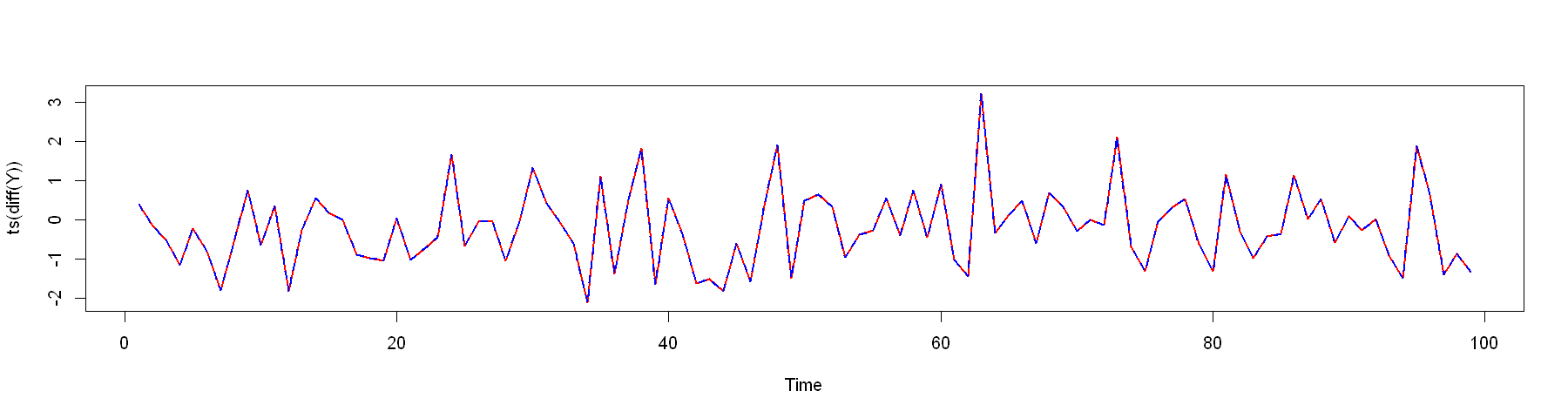So, the two models are equivalent. For simulation and forecasting we may be more interested in$Y_t$model, as it is the original series, which we are interested in. ## [INFO: END]¶ The final, 3rd model In : print(round(coef(summary(mod.3)), 4))   Estimate Std. Error t value Pr(>|t|) (Intercept) 0.0083 0.0129 0.6479 0.5173 L(lnyse) -0.0001 0.0020 -0.0471 0.9624 L(d(lnyse), 1:14)1 0.0427 0.0446 0.9574 0.3388 L(d(lnyse), 1:14)2 -0.0317 0.0447 -0.7094 0.4784 L(d(lnyse), 1:14)3 0.0198 0.0447 0.4433 0.6577 L(d(lnyse), 1:14)4 0.0217 0.0447 0.4859 0.6273 L(d(lnyse), 1:14)5 0.0957 0.0447 2.1419 0.0327 L(d(lnyse), 1:14)6 -0.0753 0.0449 -1.6792 0.0937 L(d(lnyse), 1:14)7 -0.0379 0.0449 -0.8456 0.3982 L(d(lnyse), 1:14)8 -0.0733 0.0449 -1.6329 0.1031 L(d(lnyse), 1:14)9 -0.0017 0.0449 -0.0374 0.9701 L(d(lnyse), 1:14)10 -0.0232 0.0446 -0.5202 0.6031 L(d(lnyse), 1:14)11 0.0051 0.0446 0.1139 0.9093 L(d(lnyse), 1:14)12 0.0320 0.0445 0.7184 0.4729 L(d(lnyse), 1:14)13 0.0031 0.0445 0.0688 0.9452 L(d(lnyse), 1:14)14 -0.1046 0.0445 -2.3500 0.0192  The coefficient$\widehat{\rho}$t-value is L(lnyse) = -0.0471 > -2.89. So we have no grounds to reject the null hypothesis and conclude that the series contains a unit root. We will not re-write this models equations as they can be determined by applying the same methods as before. Overall, regardless of the lag order we see that the series has a unit root. It is important to note, that removing the trend increased the t-statistic of$\widehat{\rho}$, so it is very possible that, if we incorrectly remove the trend - we may make an incorrect test conclusion. ## (3.2) Automatic Unit root testing¶ We will carry out different tests from different packages. For completeness sake, we will provide the same test results from more than one package. ### ADF test¶ The$\rm ADF$test tests the null hypothesis:$H_0: \rho = 0 \text{ (i.e. the series contains a unit root)}$We can set the maximum lag order, and choose the criterion for the order selection: In : tst_adf_1 <- urca::ur.df(lnyse, type = "trend", lags = 18, selectlags = "BIC") print(tst_adf_1)  ############################################################### # Augmented Dickey-Fuller Test Unit Root / Cointegration Test # ############################################################### The value of the test statistic is: -2.0965 6.0649 2.2091  We can examine the model In : tst_adf_1@testreg  Call: lm(formula = z.diff ~ z.lag.1 + 1 + tt + z.diff.lag) Residuals: Min 1Q Median 3Q Max -0.219561 -0.024529 0.003247 0.026918 0.152312 Coefficients: Estimate Std. Error t value Pr(>|t|) (Intercept) 9.001e-02 4.010e-02 2.244 0.0252 * z.lag.1 -1.755e-02 8.370e-03 -2.096 0.0365 * tt 1.044e-04 5.041e-05 2.070 0.0390 * z.diff.lag 5.043e-02 4.444e-02 1.135 0.2570 --- Signif. codes: 0 '***' 0.001 '**' 0.01 '*' 0.05 '.' 0.1 ' ' 1 Residual standard error: 0.0409 on 506 degrees of freedom Multiple R-squared: 0.01033, Adjusted R-squared: 0.004462 F-statistic: 1.76 on 3 and 506 DF, p-value: 0.1538  The critical values can be extracted via @cval: In : print(tst_adf_1@cval)   1pct 5pct 10pct tau3 -3.96 -3.41 -3.12 phi2 6.09 4.68 4.03 phi3 8.27 6.25 5.34  To carry out the unit root test, we examine the t-statistic of z.lag.1 - it is -2.096 (Note that this is the same as the first value in the output The value of the test statistic is: -2.0965 6.0649 2.2091). The relevant critival value is tau, which is -3.41 for the$5\%$significance level. Since The test statistic -2.096 > -3.45, we do not reject the null hypothesis of a unit root. The second way to carry out and$\rm ADF$test: In : tst_adf_2 <- tseries::adf.test(lnyse, alternative = "stationary")  We can either manually specify the lag order, or let the function select the maximum lag order itself. In : tst_adf_2   Augmented Dickey-Fuller Test data: lnyse Dickey-Fuller = -1.9019, Lag order = 8, p-value = 0.6198 alternative hypothesis: stationary  This output is a bit easier to read - the$p-value = 0.6198 > 0.05$, so we have no grounds to reject the null hypothesis of a unit root. ### KPSS test¶ The$\rm KPSS$test tests the null hypothesis:$H_0: \text{ the data is stationary}$The test involves specifying and estimating the following equation with a random-walk component: $$Y_t = r_t + \delta \cdot t + \epsilon_t,\quad r_t = r_{t-1} + u_t$$ The null hypothesis of stationarity is equivalent to the hypothesis:$H_0: \sigma^2_u = 0$, which would mean that$r_t = r_{t-1} = ... = r_0 = \rm const$(i.e. a constant intercept). We can set the number of lags to use the rule of thumb (lags = "long", which is equivalent to$\root 4 \of {12 \times (n/100)}$), or lags = "short", which is equivalent to$\root 4 \of {4 \times (n/100)}$. The test types specify as deterministic component either a constant mu or a constant with linear trend tau. In : tst_kpss_1 <- urca::ur.kpss(lnyse, type = "tau", lags = "long") tst_kpss_1  ####################################### # KPSS Unit Root / Cointegration Test # ####################################### The value of the test statistic is: 0.3909  We see that the test statistic is 0.3909. The critical values are: In : tst_kpss_1@cval  10pct5pct2.5pct1pct critical values0.1190.1460.1760.216 Since 0.3909 > 0.146, we reject the null hypothesis of stationarity and conclude that the series is not stationary. The second way to carry out the KPSS test (where lshort = FALSE indicates to use the rule-of-thumb larger lag selection): In : tst_kpss_2 <- tseries::kpss.test(lnyse, null = "Trend", lshort = FALSE)  Warning message in tseries::kpss.test(lnyse, null = "Trend", lshort = FALSE): "p-value smaller than printed p-value" In : print(tst_kpss_2)   KPSS Test for Trend Stationarity data: lnyse KPSS Trend = 0.43099, Truncation lag parameter = 16, p-value = 0.01  Since p-value = 0.01 < 0.05, we reject the null hypothesis of stationarity and conclude that the series is not stationary. ### PP test¶ The$\rm PP$test tests builds on the ADF test and tests the null hypothesis:$H_0: \text{ (i.e. the series contains a unit root)}$. We begin with the built-in function: In : tst_pp_0 <- stats::PP.test(lnyse, lshort = FALSE)  In : tst_pp_0   Phillips-Perron Unit Root Test data: lnyse Dickey-Fuller = -1.9395, Truncation lag parameter = 18, p-value = 0.6039  p-value = 0.6039 > 0.05, so we have no grounds to reject the null hypothesis of a unit root. The urca package also contains the$PP$test: In : tst_pp_1 <- urca::ur.pp(lnyse, model = "trend", type = "Z-tau", lags = "long") tst_pp_1  ################################################## # Phillips-Perron Unit Root / Cointegration Test # ################################################## The value of the test statistic is: -1.9393  Note: use type = "Z-tau", otherwise the critical values will be missing!. If we are interested, we can examine the specified regression, which was used for this test: In : tst_pp_1@testreg  Call: lm(formula = y ~ y.l1 + trend) Residuals: Min 1Q Median 3Q Max -0.221927 -0.025066 0.002876 0.026943 0.147915 Coefficients: Estimate Std. Error t value Pr(>|t|) (Intercept) 1.045e-01 5.145e-02 2.032 0.0427 * y.l1 9.845e-01 8.151e-03 120.792 <2e-16 *** trend 9.600e-05 4.958e-05 1.936 0.0534 . --- Signif. codes: 0 '***' 0.001 '**' 0.01 '*' 0.05 '.' 0.1 ' ' 1 Residual standard error: 0.04055 on 525 degrees of freedom Multiple R-squared: 0.9981, Adjusted R-squared: 0.9981 F-statistic: 1.38e+05 on 2 and 525 DF, p-value: < 2.2e-16  Although the critical test statistic is calculated differently than the one in the$ADF$test, the critical values for the PP test are the same as for the DF test. The critical values are: In : tst_pp_1@cval  1pct5pct10pct critical values-3.97979 -3.420314-3.132507 Since the value of the test statistic -1.9393 is greater than the$5\%$critical value -3.420314, we have no grounds to reject the null hypothesis of a unit root. The tseries package also has the$PP$test: In : tst_pp_2 <- tseries::pp.test(lnyse, alternative = "stationary", type = "Z(t_alpha)", lshort = FALSE)  In : tst_pp_2   Phillips-Perron Unit Root Test data: lnyse Dickey-Fuller Z(t_alpha) = -1.9395, Truncation lag parameter = 18, p-value = 0.6039 alternative hypothesis: stationary  The p-value = 0.6039 > 0.05, we have no grounds to reject the null hypothesis and conclude that the series has a unit root. So, we can conclude that all tests indicate that the series has a unit root. In : # Set plot options in Jupyter Lab options(repr.plot.width = 10) options(repr.plot.height = 4)  # (4) Model Building¶ Since we have determined that the series has a unit root (and we see no indication of seasonality), we can take the differences of the series: In : dlnyse <- diff(lnyse)  If we examine the series: In : forecast::tsdisplay(dlnyse)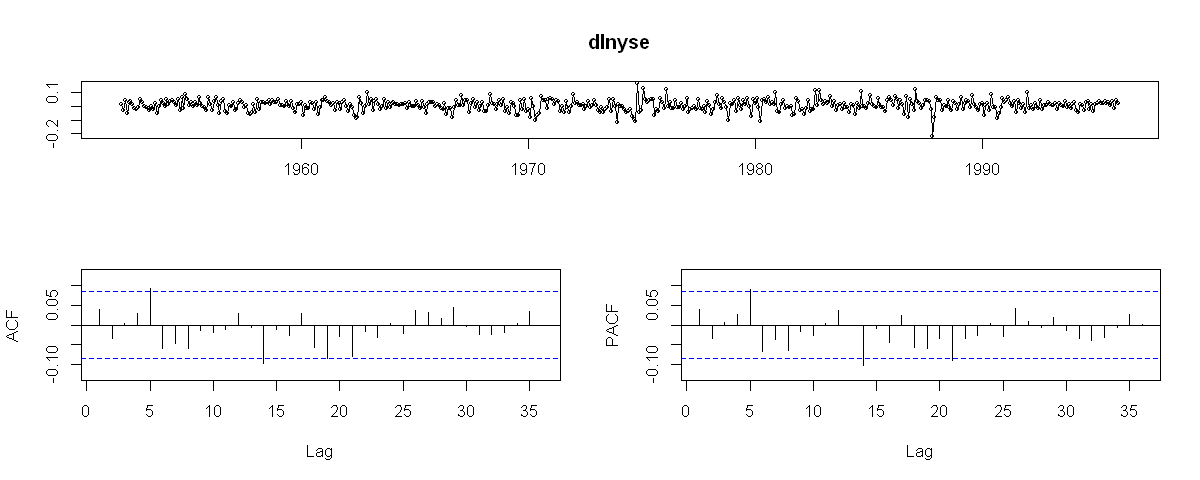The series appear to be stationary. We will test this by repeating the unit root tests on the differenced data. For simplicity, we will use the test, which have the p-values, from the tseries package: In : tseries::adf.test(dlnyse, alternative = "stationary")  Warning message in tseries::adf.test(dlnyse, alternative = "stationary"): "p-value smaller than printed p-value"  Augmented Dickey-Fuller Test data: dlnyse Dickey-Fuller = -8.1328, Lag order = 8, p-value = 0.01 alternative hypothesis: stationary $p-value = 0.01 < 0.05$, so we reject the null hypothesis of a unit root. In : tseries::kpss.test(dlnyse, null = "Level", lshort = FALSE)  Warning message in tseries::kpss.test(dlnyse, null = "Level", lshort = FALSE): "p-value greater than printed p-value"  KPSS Test for Level Stationarity data: dlnyse KPSS Level = 0.095985, Truncation lag parameter = 16, p-value = 0.1 $p-value = 0.1 > 0.05$, so we have no grounds to reject the null hypothesis of stationarity. In : tseries::pp.test(dlnyse, alternative = "stationary", type = "Z(t_alpha)", lshort = FALSE)  Warning message in tseries::pp.test(dlnyse, alternative = "stationary", type = "Z(t_alpha)", : "p-value smaller than printed p-value"  Phillips-Perron Unit Root Test data: dlnyse Dickey-Fuller Z(t_alpha) = -22.021, Truncation lag parameter = 18, p-value = 0.01 alternative hypothesis: stationary $p-value = 0.01 < 0.05$, so we reject the null hypothesis of a unit root. In other words, the differences,$\Delta Y_t$, are stationary. To make it interesting, we will specify three different models: • A model based on one selected from the$\rm ADF$test; • A model based on the$\rm ACF$,$\rm PACF$of$\Delta Y_t$; • An automatically specified model via auto.arima, without any restrictions. ## - From the ADF test we select and re-estimate mod.2 model (this is a different parametrization than the one on dynlm)¶ Furthermore, we will only be able to estimate the drift component, since if$Y_t$contais a constant$\alpha$and trend$\delta \cdot t$, then$\Delta Y_t$contains$\delta$. In this specification$\alpha$is not identifiable. In : mdl_4_1 <- forecast::Arima(lnyse, order = c(5, 1, 0), xreg = data.frame(tt = 1:length(lnyse)))  In : print(mdl_4_1)  Series: lnyse Regression with ARIMA(5,1,0) errors Coefficients: ar1 ar2 ar3 ar4 ar5 tt 0.0380 -0.0350 0.0089 0.0228 0.0923 0.0069 s.e. 0.0433 0.0434 0.0434 0.0434 0.0434 0.0020 sigma^2 estimated as 0.001645: log likelihood=945.98 AIC=-1877.96 AICc=-1877.75 BIC=-1848.08  The residuals of this model: In : forecast::tsdisplay(mdl_4_1$residuals)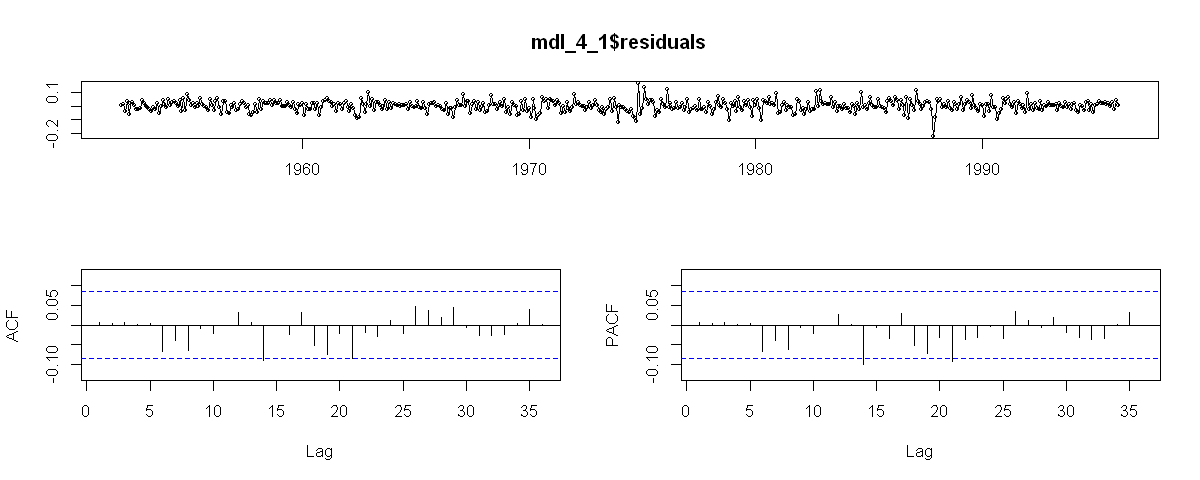Appear to be white noise.

## [INFO: START]¶

We will now expand upon what a different parametrization entails for models specified dynlm versus the ones specified with Arima().

#### - The model, specified via forecast::Arima (or via stats::arima) is specified as a process with a linear trend, whose deviations from the trend are described as the AR(1) process:¶

For example, assume that we would want to specify a model as a stationary $\rm AR(1)$ model with a trend using forecast::Arima (or stats::arima) the specification is as follows:

$\begin{cases} Y_t &= \alpha + \delta \cdot t + u_t,\\ u_t &= \phi u_{t-1} + \epsilon_t \end{cases}$

In :
mod.a1 <- stats::arima(lnyse, order = c(1,0,0), xreg=time(lnyse))
mod.a1

Call:
stats::arima(x = lnyse, order = c(1, 0, 0), xreg = time(lnyse))

Coefficients:
ar1  intercept  time(lnyse)
0.9832  -144.2528       0.0763
s.e.  0.0076    12.4969       0.0063

sigma^2 estimated as 0.001632:  log likelihood = 945.18,  aic = -1882.37
In :
mod.a2 <- forecast::Arima(lnyse, order = c(1,0,0), xreg=time(lnyse))
mod.a2

Series: lnyse
Regression with ARIMA(1,0,0) errors

Coefficients:
ar1  intercept  time(lnyse)
0.9832  -144.2528       0.0763
s.e.  0.0076    12.4969       0.0063

sigma^2 estimated as 0.001642:  log likelihood=945.18
AIC=-1882.37   AICc=-1882.29   BIC=-1865.28

The estimated model is:

$\begin{cases} Y_t &= -144.2528 + 0.0763 \cdot t + u_t,\\ u_t &= 0.9832 u_{t-1} + \epsilon_t \end{cases}$

In this case, we would want to test whether the residuals exhibit a unit root by testing whether $\phi = 1$.

#### - The model, specified via dynlm::dynlm is specified as an autoregressive process with a linear trend:¶

From the (1) expression we would have that:

$\begin{cases} u_t &= Y_t - \alpha - \delta \cdot t,\\ u_t &= \phi u_{t-1} + \epsilon_t \end{cases} \Longrightarrow Y_t - \alpha - \delta \cdot t = \phi \left(Y_{t-1} - \alpha - \delta \cdot (t-1) \right) + \epsilon_t$

which means that an alternative specification involves a reparametrization of the previous process as:

\begin{aligned} Y_t &= \tilde{\alpha} + \tilde{\delta} \cdot t + \phi Y_{t-1} + \epsilon_t = \left[ (1 - \phi) \alpha + \phi \delta\right] + \left[ (1 - \phi)\delta \right] \cdot t + \phi Y_{t-1} + \epsilon_t \end{aligned}

which is as an autoregressive process with a linear trend.

This is the specification that dynlm::dynlm(...) uses:

In :
mod.b1 <- dynlm::dynlm(lnyse ~ time(lnyse) + L(lnyse))
summary(mod.b1)

Time series regression with "ts" data:
Start = 1952(2), End = 1996(1)

Call:
dynlm::dynlm(formula = lnyse ~ time(lnyse) + L(lnyse))

Residuals:
Min        1Q    Median        3Q       Max
-0.221927 -0.025066  0.002876  0.026943  0.147915

Coefficients:
Estimate Std. Error t value Pr(>|t|)
(Intercept) -2.169524   1.124569  -1.929   0.0542 .
time(lnyse)  0.001152   0.000595   1.936   0.0534 .
L(lnyse)     0.984518   0.008150 120.792   <2e-16 ***
---
Signif. codes:  0 '***' 0.001 '**' 0.01 '*' 0.05 '.' 0.1 ' ' 1

Residual standard error: 0.04055 on 525 degrees of freedom
Multiple R-squared:  0.9981,	Adjusted R-squared:  0.9981
F-statistic: 1.38e+05 on 2 and 525 DF,  p-value: < 2.2e-16


The estimated model is:

\begin{aligned} Y_t &= 2.169524 + 0.001152 \cdot t + 0.984518 Y_{t-1} + \epsilon_t \end{aligned}

The models are equivalent, but due to the parametrization $\alpha \neq \tilde \alpha$ and $\delta \neq \tilde{\delta}$. The autoregressive coefficient $\widehat{\phi}$ also differ due to different parameter estimation methods (which again may further differ for different parametrization options). However they are fairly close: ar1 = 0.9832 versus L(lnyse) = 0.984518.

If we use the parametrization on the estimated coefficients from the first model, we would have that:

\begin{aligned} \tilde{\alpha} &= \left[ (1 - \phi) \alpha + \phi \delta\right]\\ \tilde{\delta} &=\left[ (1 - \phi)\delta \right] \end{aligned}

In :
# tilde{alpha}
(1 - 0.9832) * (-144.2528) + 0.9832 * 0.0763

-2.34842888000001
In :
# tilde{delta}
(1 - 0.9832) * 0.0763

0.00128184

We see that they are fairly close to (Intercept) = -2.169524 and time(lnyse) = 0.001152 respectively.

## - Selecting the model based on the ACF and PACF of the series:¶

In :
par(mfrow = c(1, 2))
forecast::Acf(diff(lnyse))
forecast::Pacf(diff(lnyse))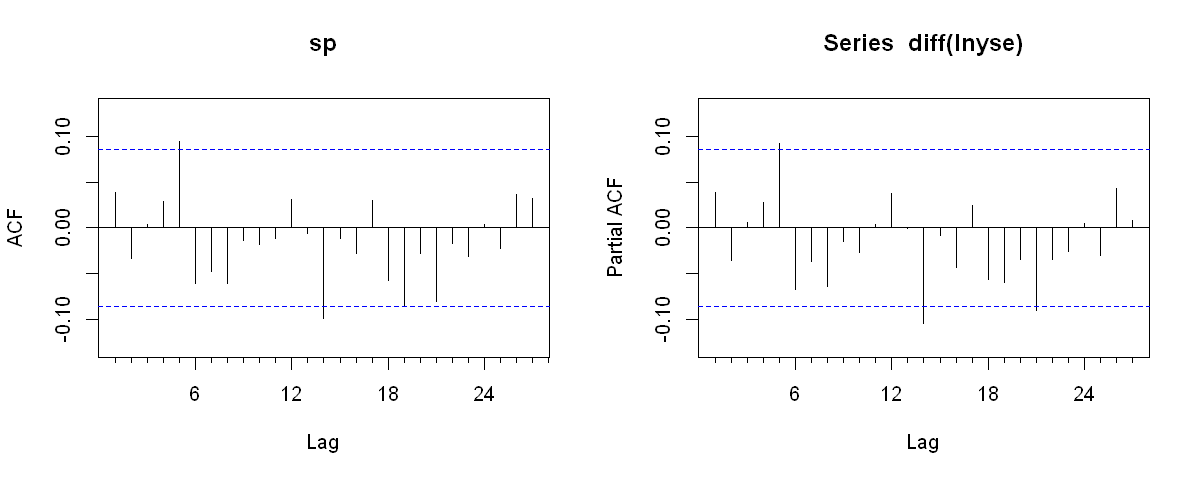We can test the lag significance via the $Ljung-Box$ test. The null hypothesis is: \begin{aligned} H_0&: \rho(1) = ... = \rho(k) = 0\\ H_1&: \exists j: \rho(j) \neq 0 \end{aligned} We will test, whether the first 20 lags have a significant autocorrelation with different values of $k$:

In :
for(i in 1:20){
aa <- Box.test(diff(lnyse), lag = i, type = "Ljung-Box")
print(paste0("lag = ", i, " p-value = ", round(aa$p.value, 5))) }   "lag = 1 p-value = 0.36736"  "lag = 2 p-value = 0.49395"  "lag = 3 p-value = 0.70145"  "lag = 4 p-value = 0.76131"  "lag = 5 p-value = 0.25479"  "lag = 6 p-value = 0.20216"  "lag = 7 p-value = 0.20613"  "lag = 8 p-value = 0.1685"  "lag = 9 p-value = 0.2293"  "lag = 10 p-value = 0.29176"  "lag = 11 p-value = 0.36542"  "lag = 12 p-value = 0.40789"  "lag = 13 p-value = 0.48727"  "lag = 14 p-value = 0.21937"  "lag = 15 p-value = 0.27295"  "lag = 16 p-value = 0.31138"  "lag = 17 p-value = 0.34642"  "lag = 18 p-value = 0.30703"  "lag = 19 p-value = 0.17814"  "lag = 20 p-value = 0.20485"  We see that in all cases the$p-value > 0.05$, so we have no grounds to reject the null hypothesis. This indicates that the differences,$\Delta Y_t$, are white noise. We can specify the model as: In : mdl_4_2 <- forecast::Arima(lnyse, order = c(0, 1, 0), include.drift = TRUE)  In : print(mdl_4_2)  Series: lnyse ARIMA(0,1,0) with drift Coefficients: drift 0.0069 s.e. 0.0018 sigma^2 estimated as 0.00165: log likelihood=942.79 AIC=-1881.59 AICc=-1881.56 BIC=-1873.05  The residuals In : forecast::tsdisplay(mdl_4_2$residuals)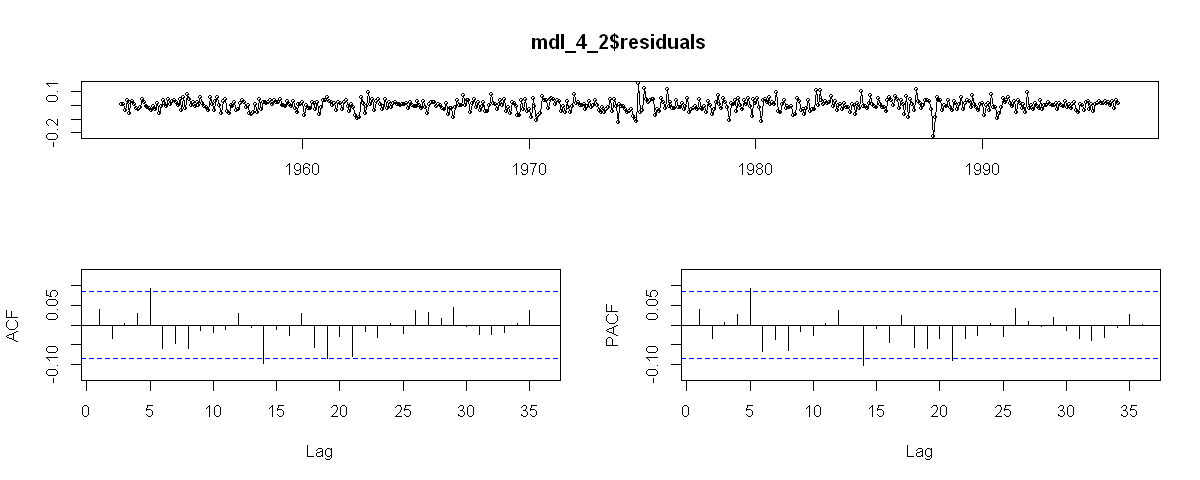In :
for(i in (length(coef(mdl_4_2)) + 1):`